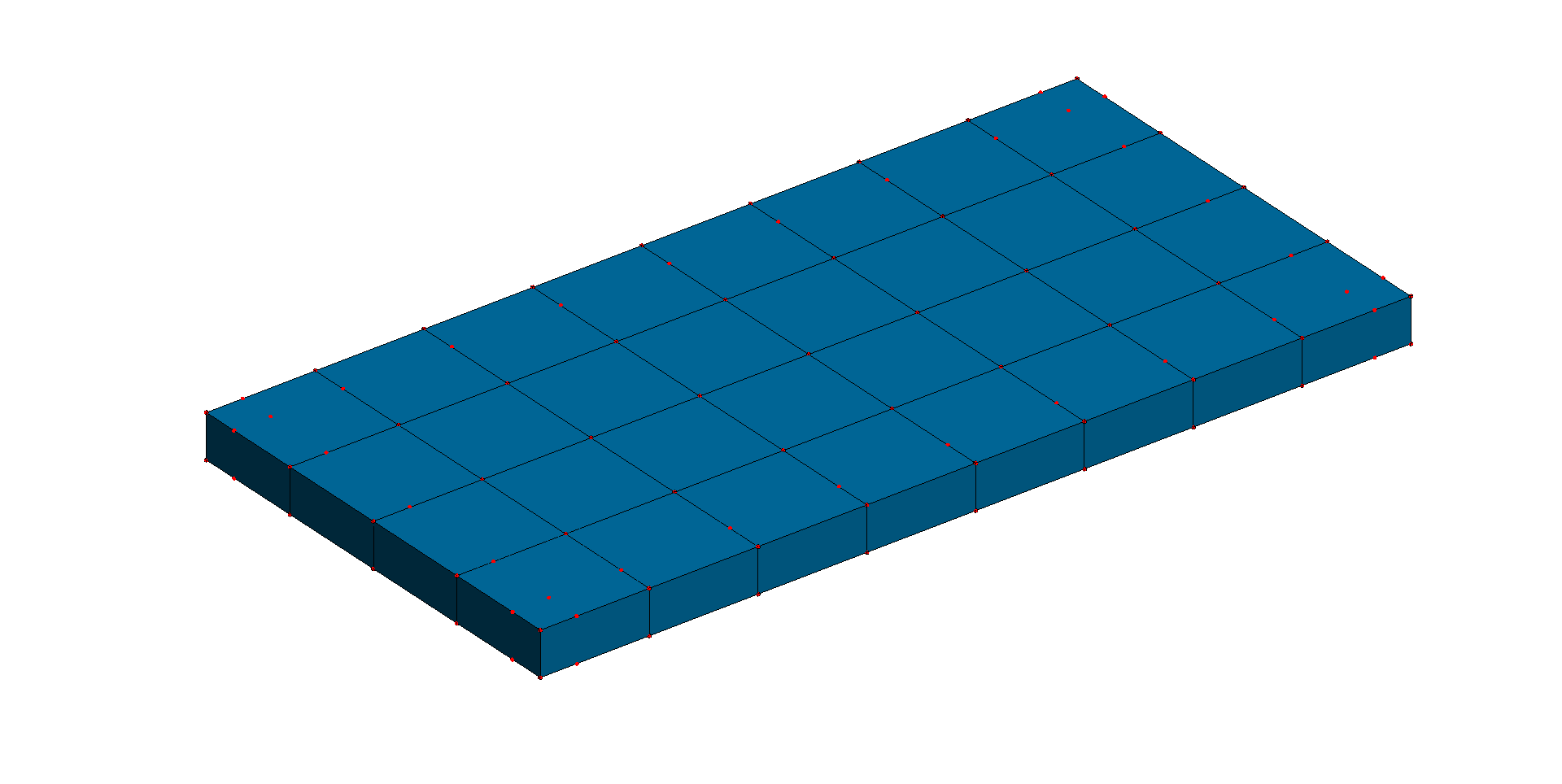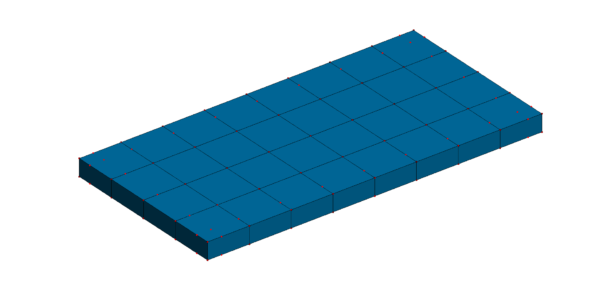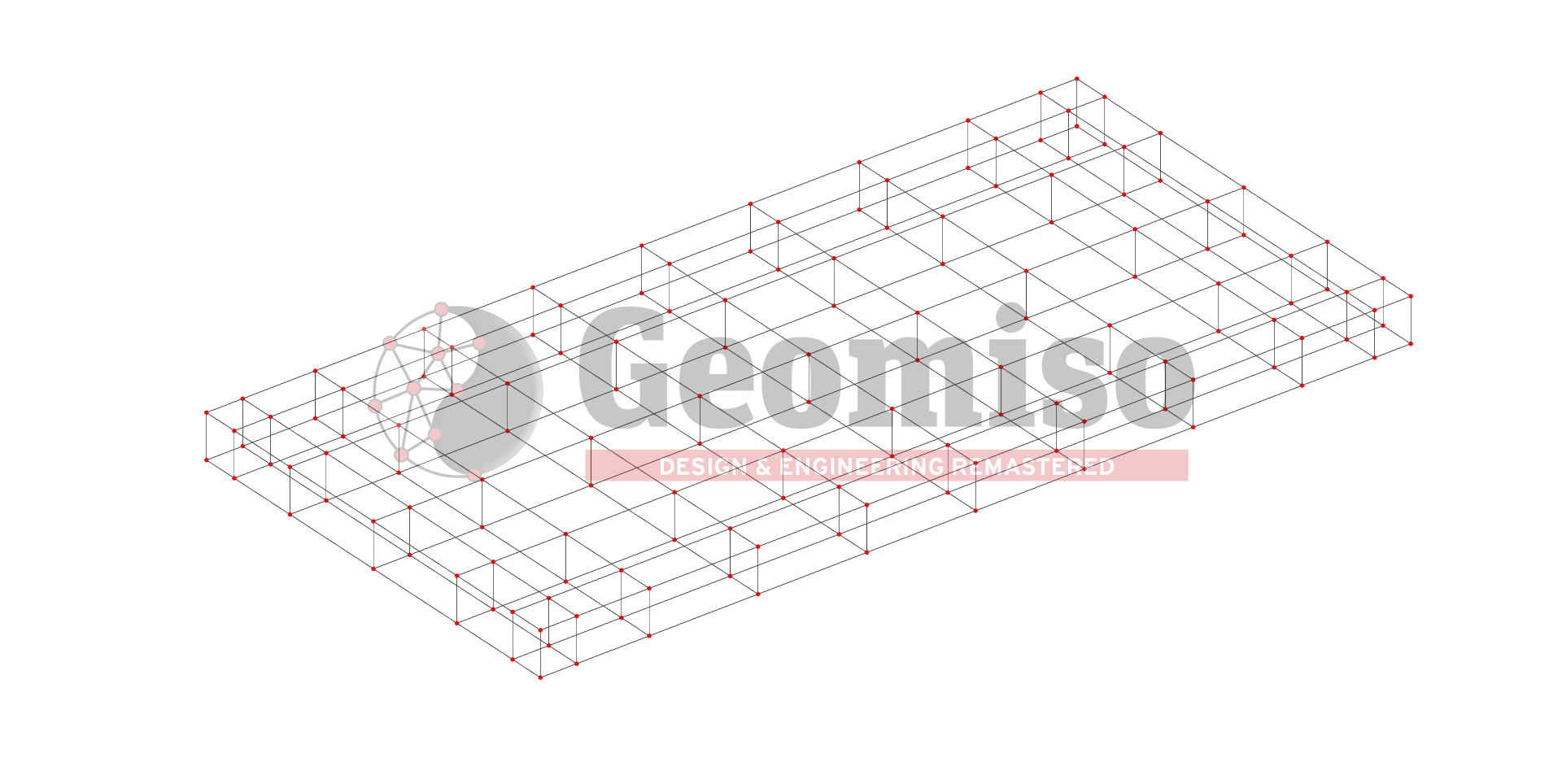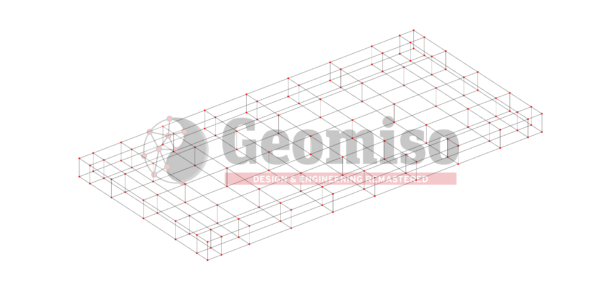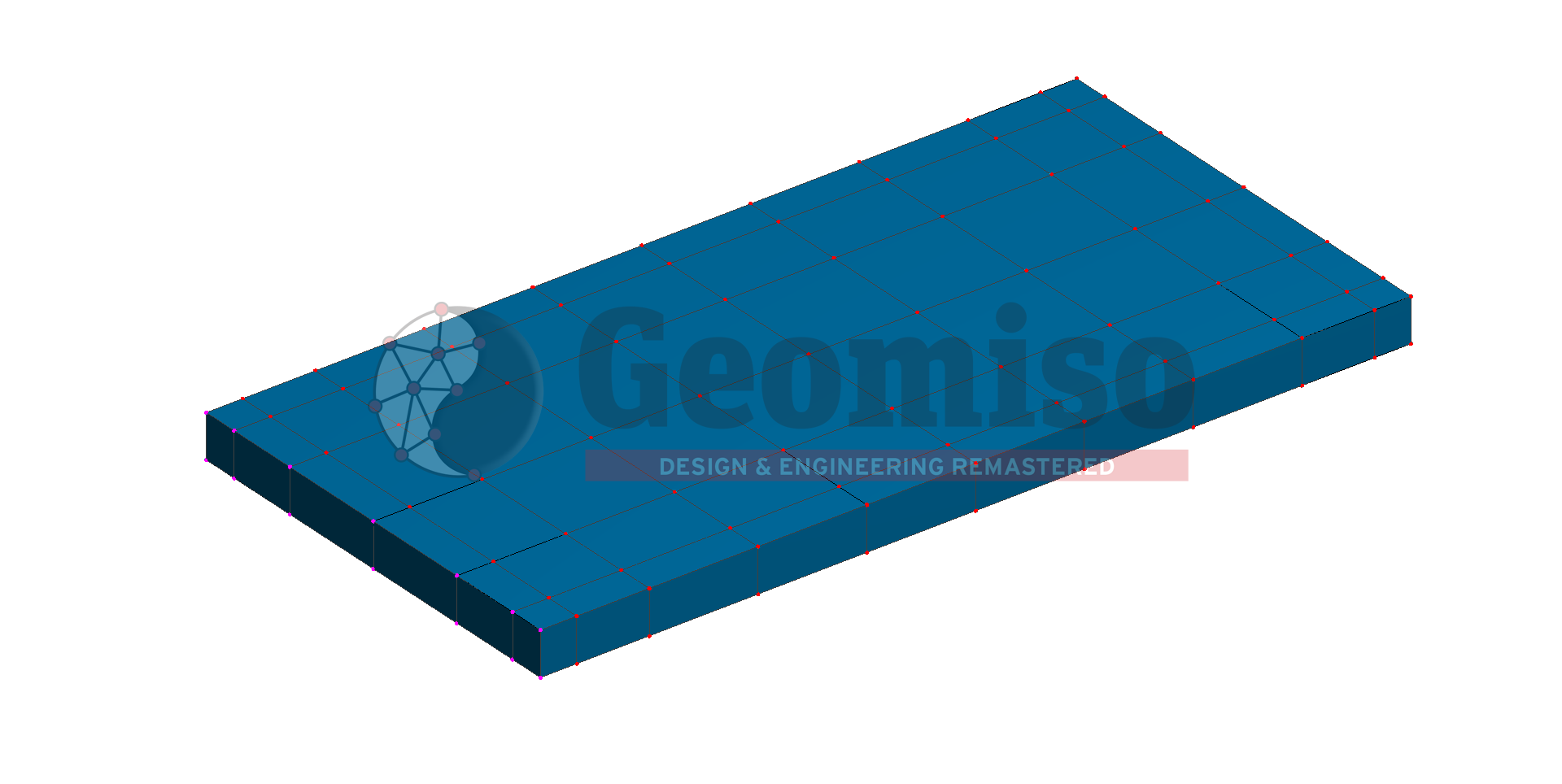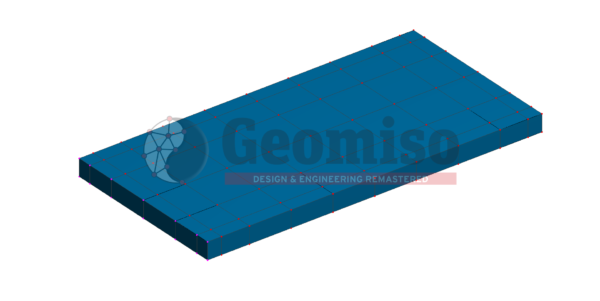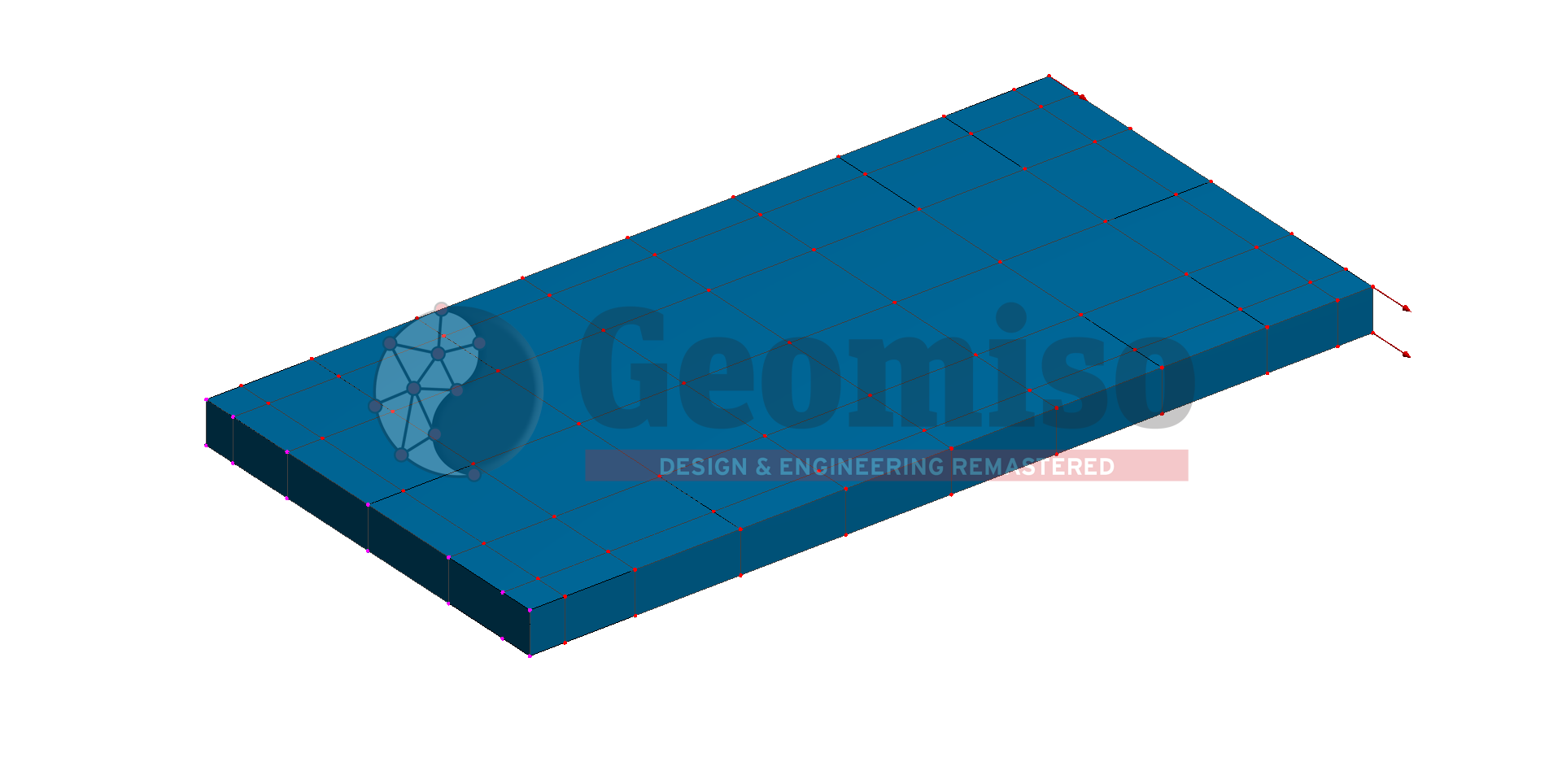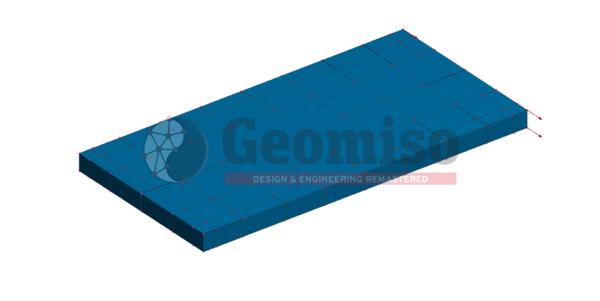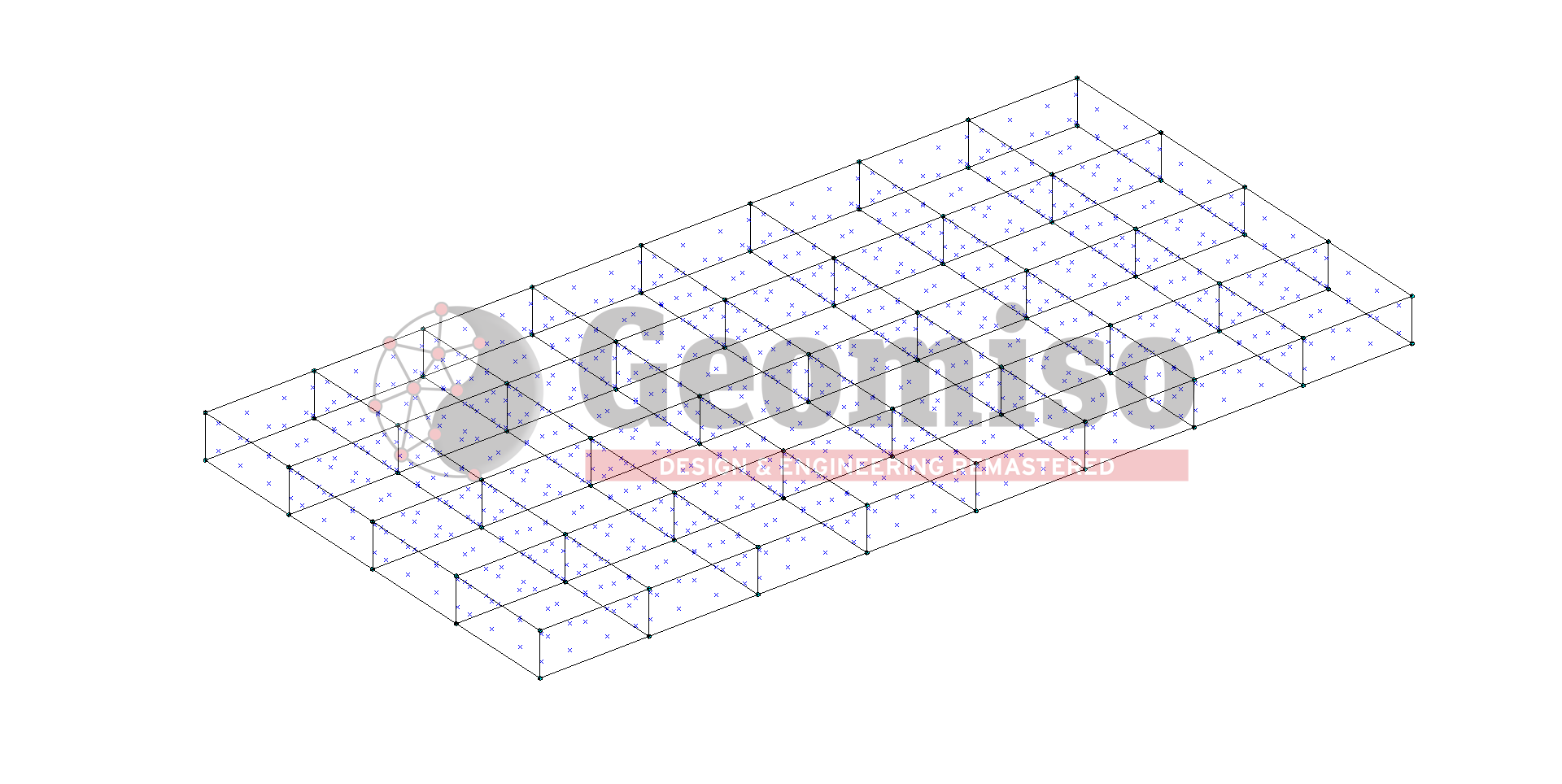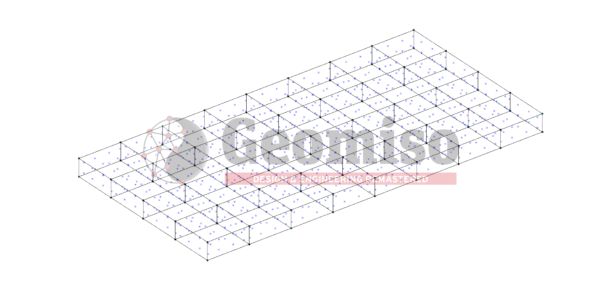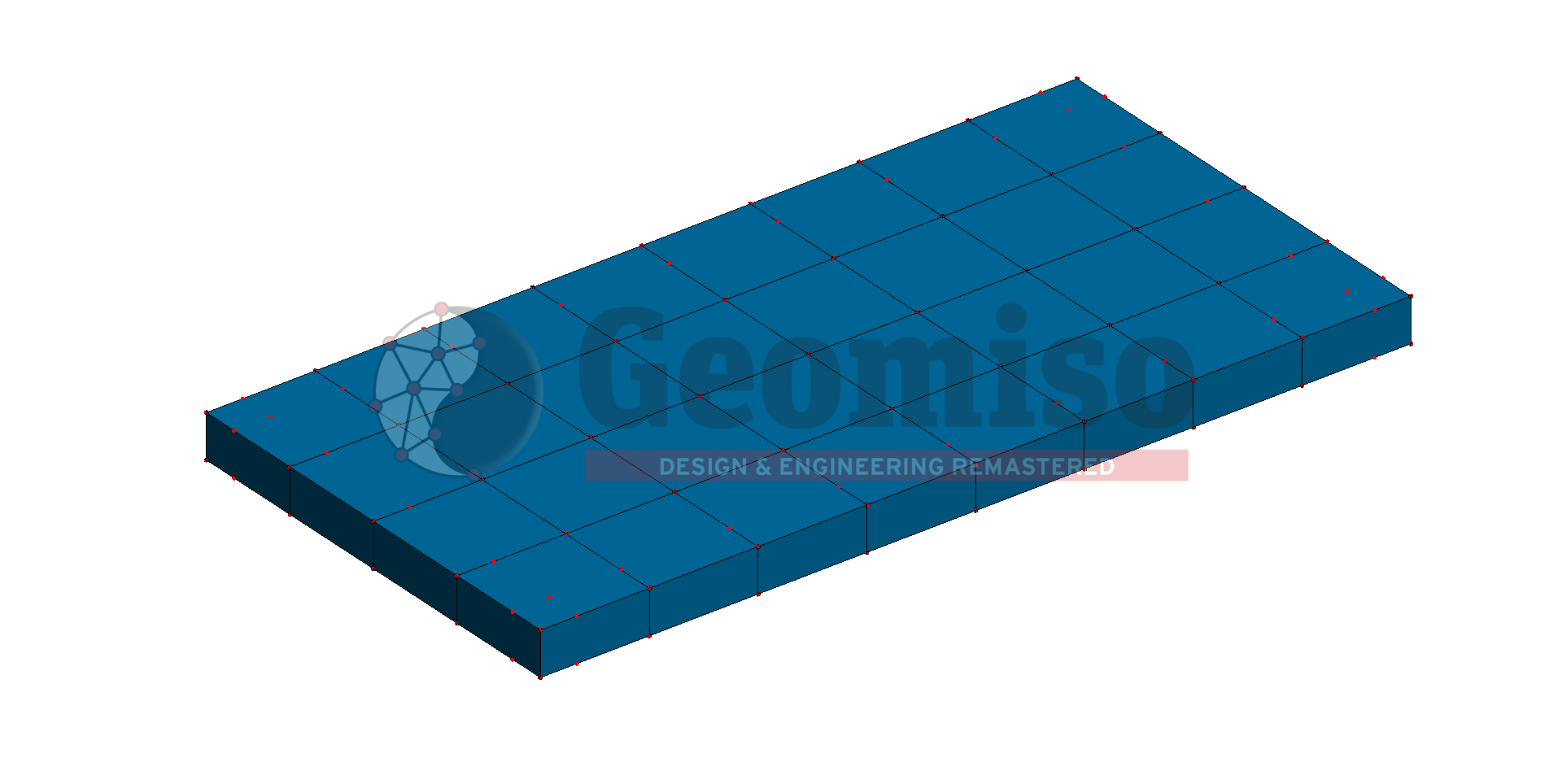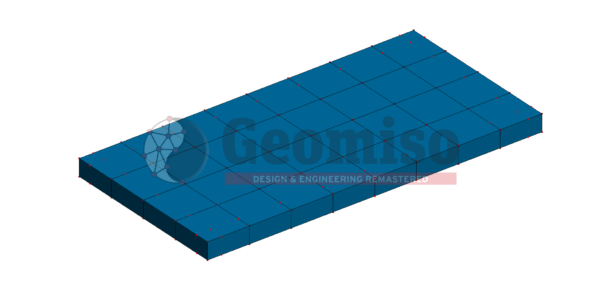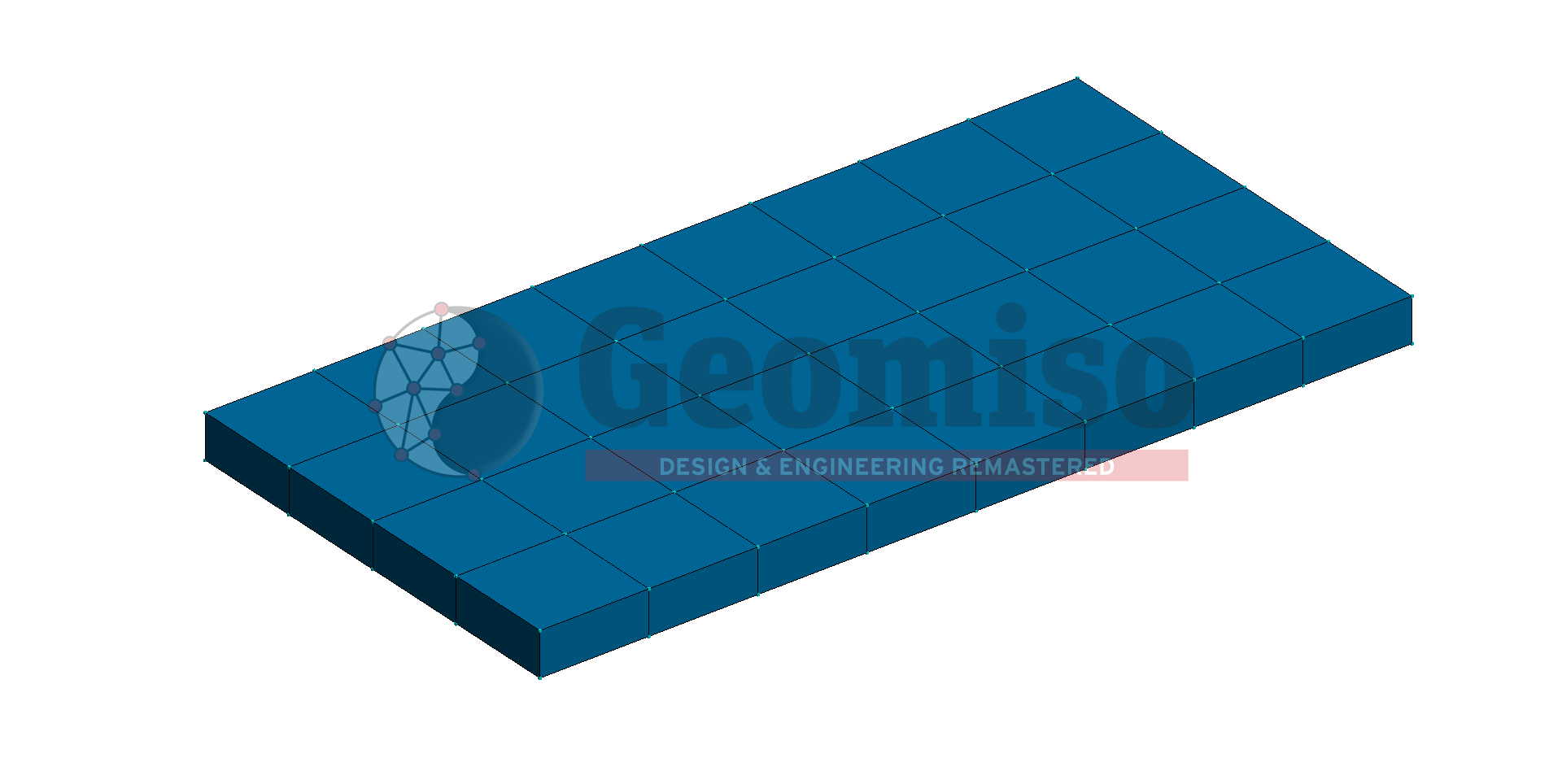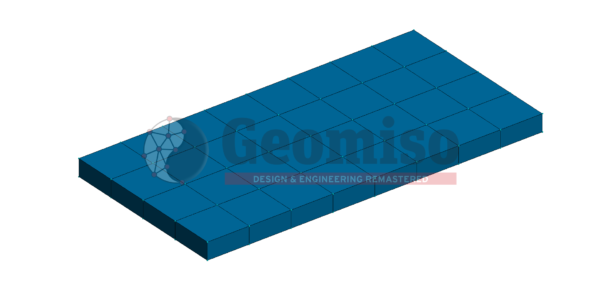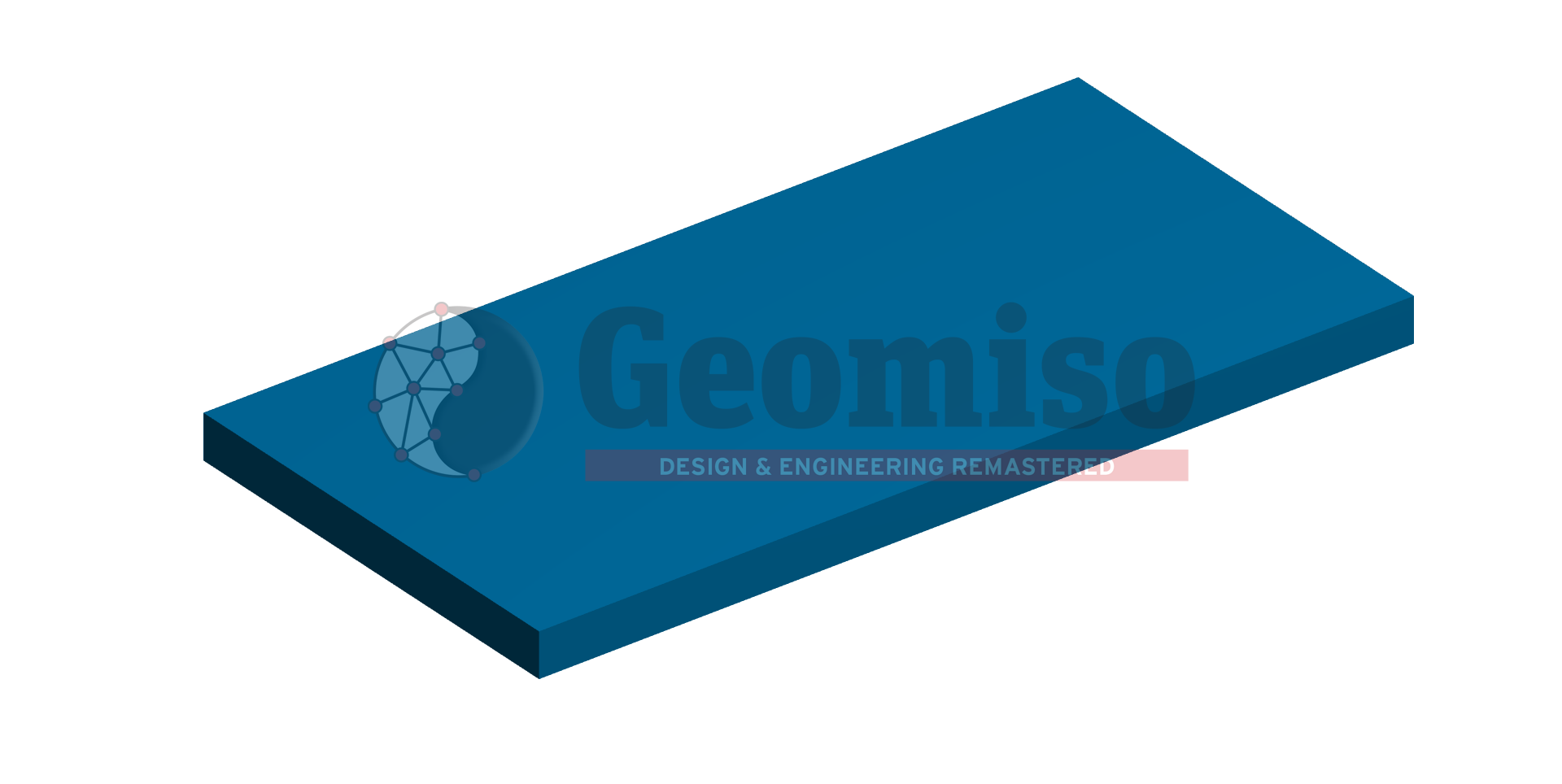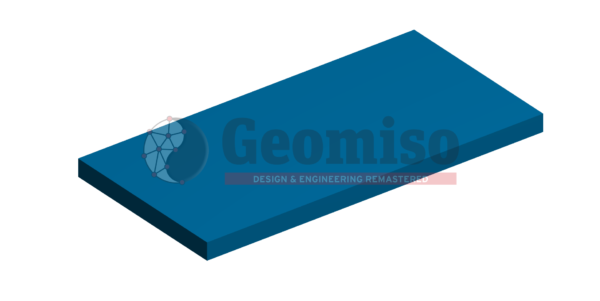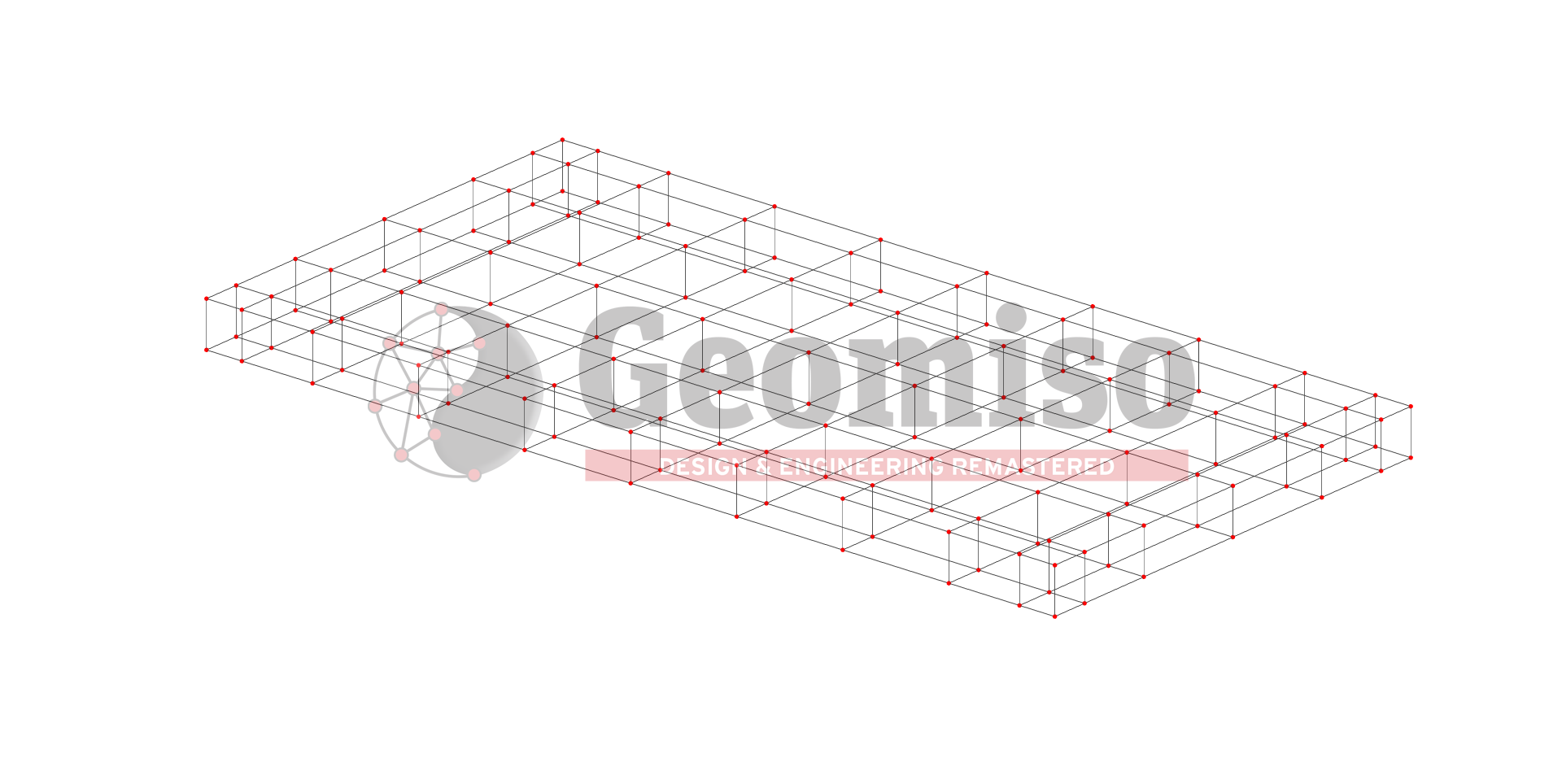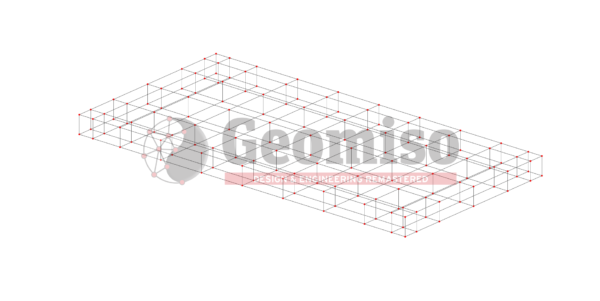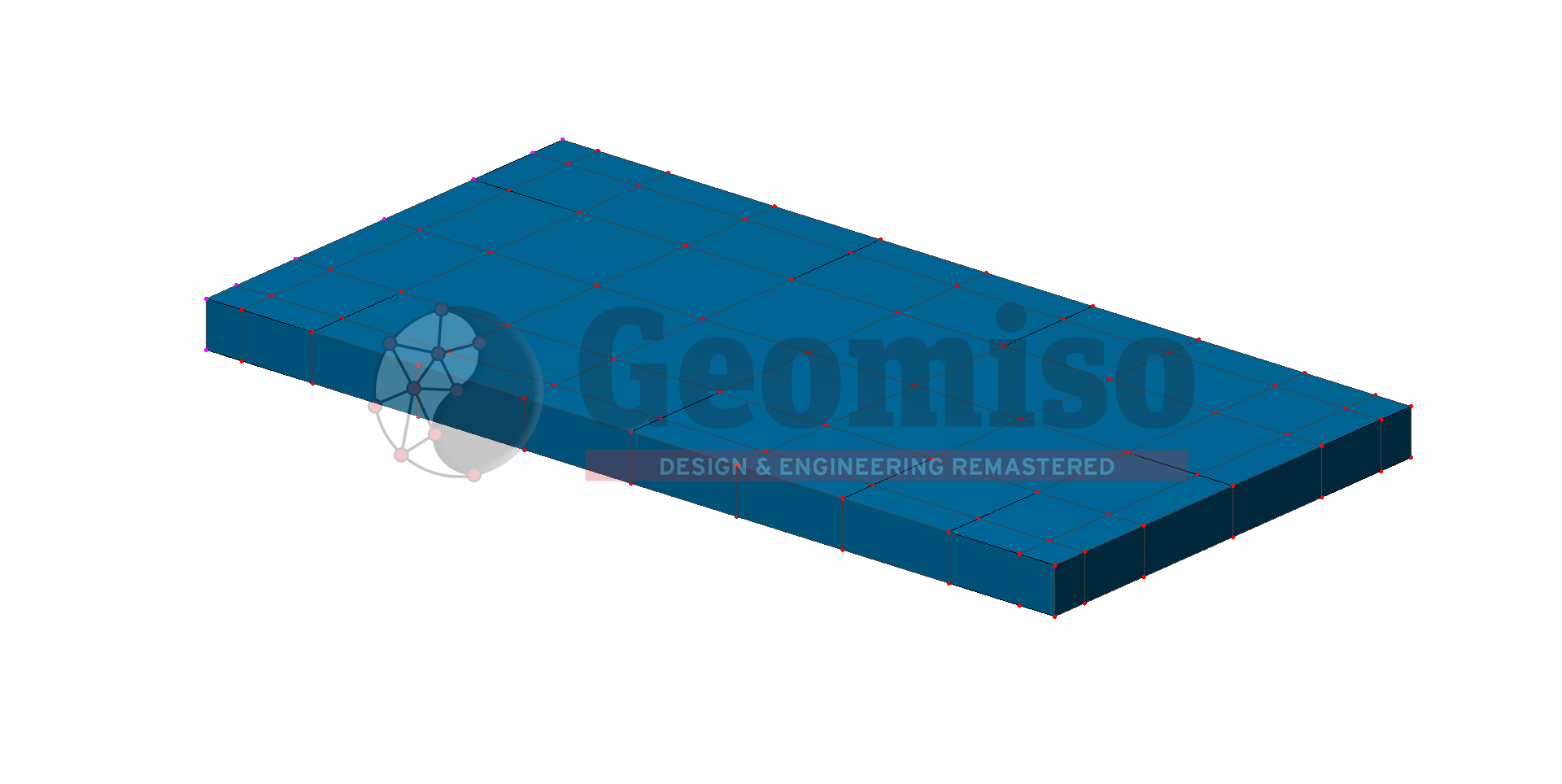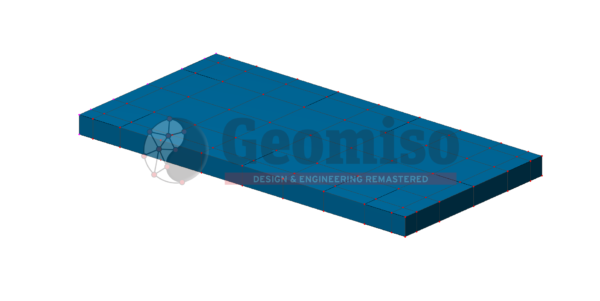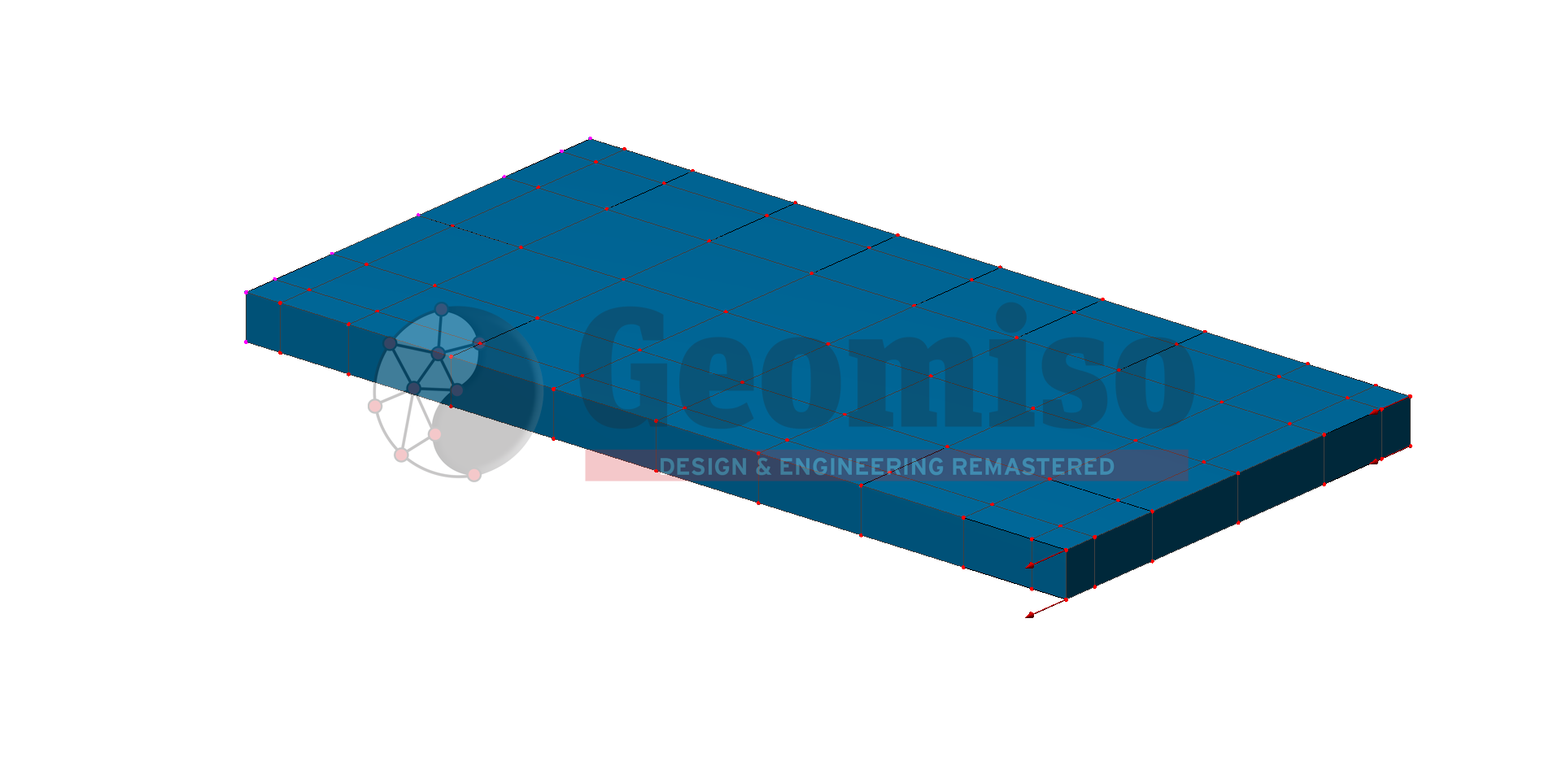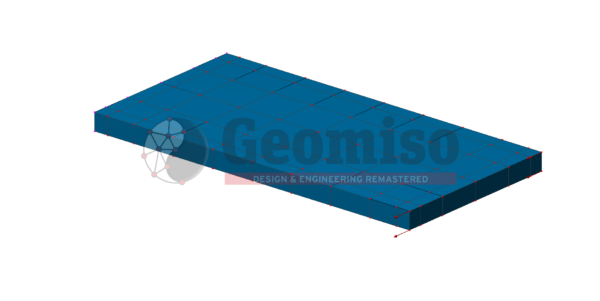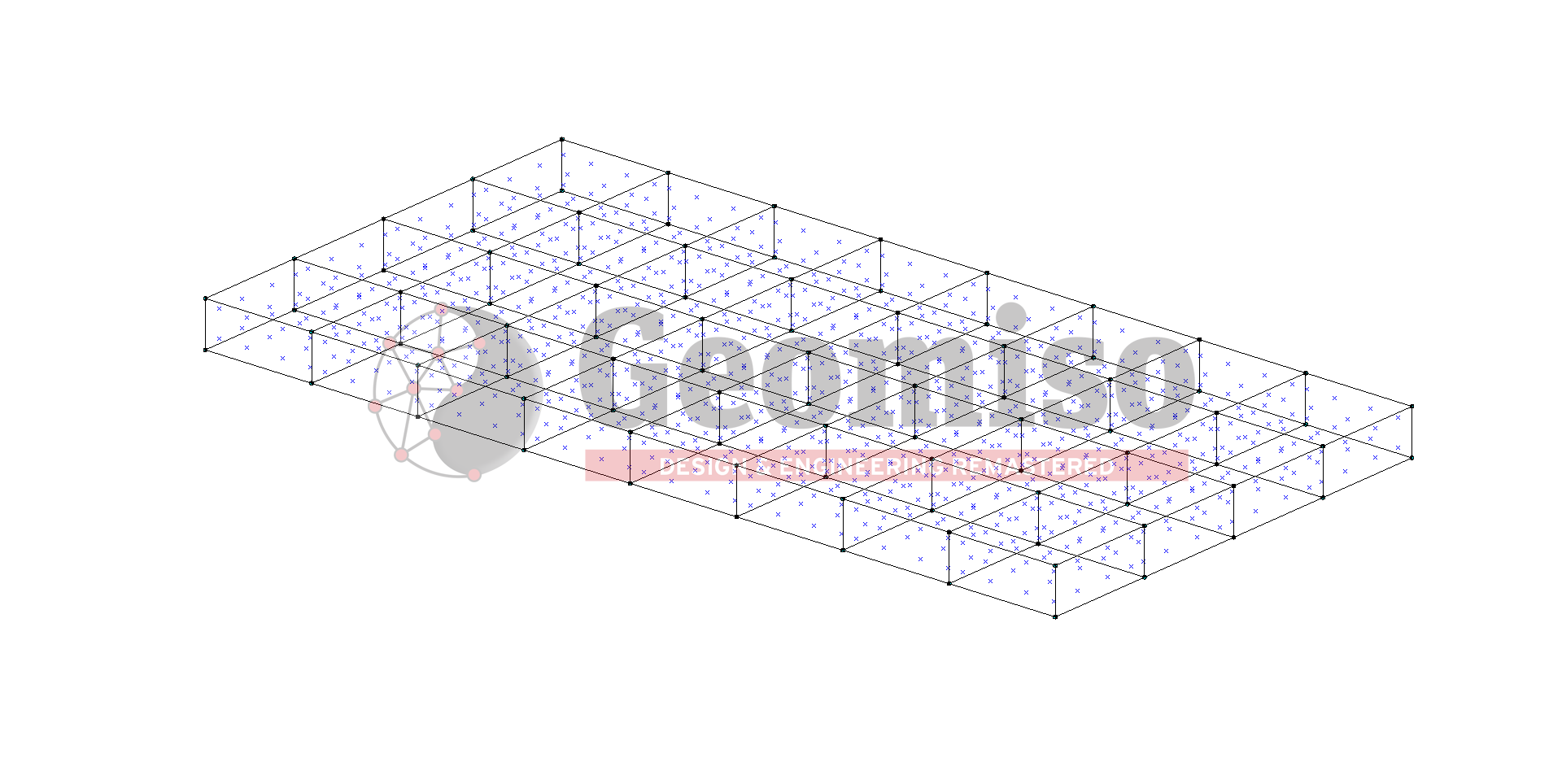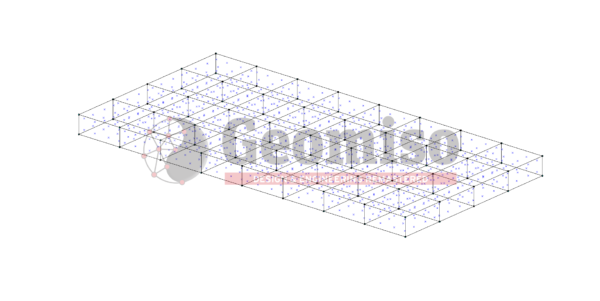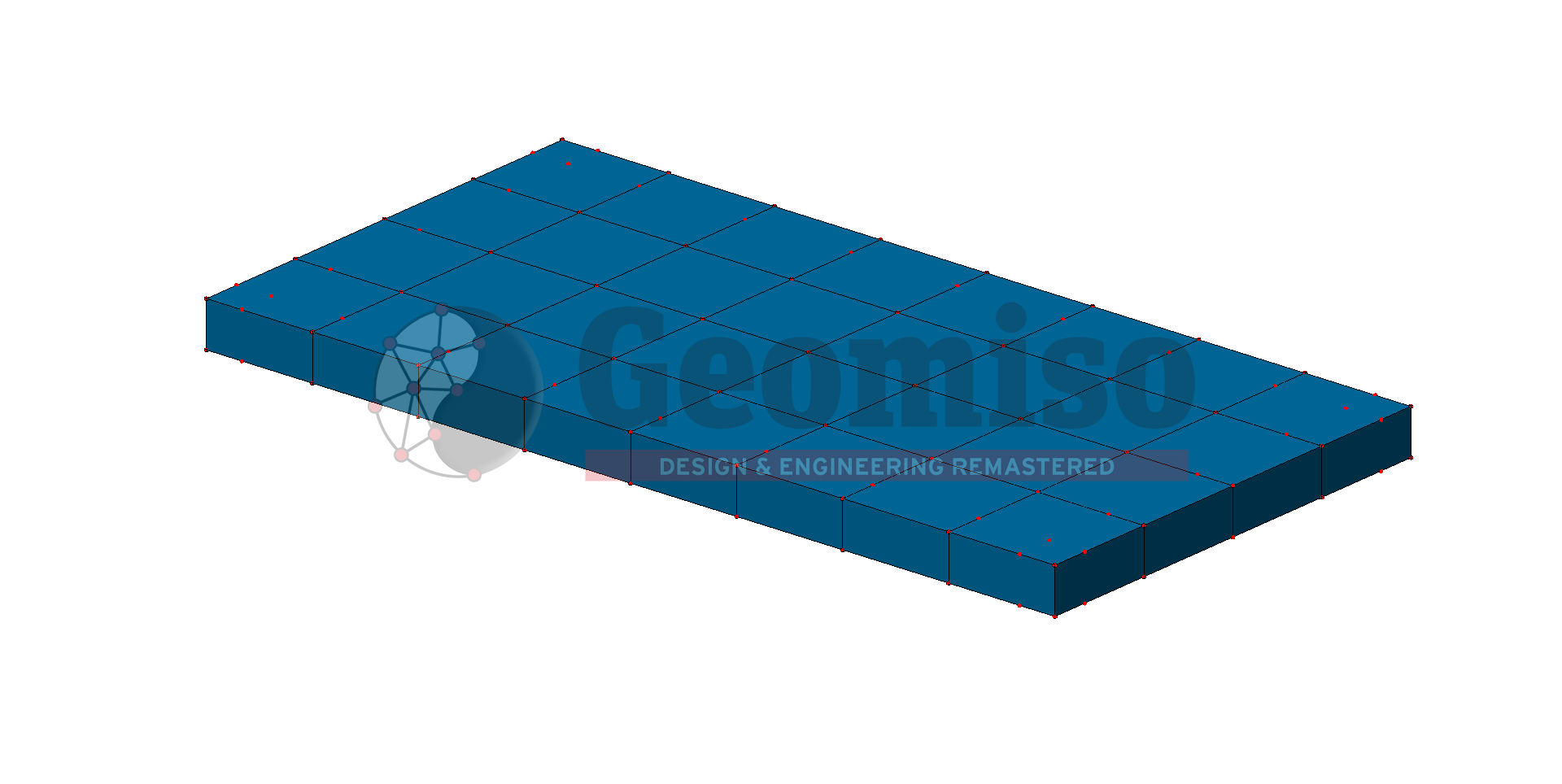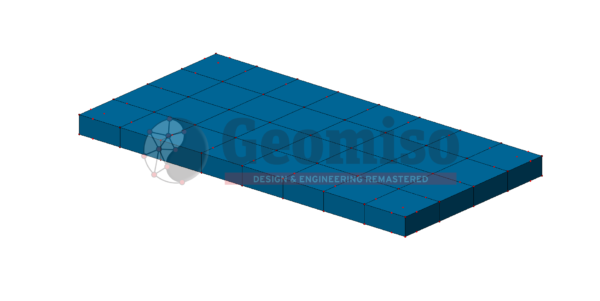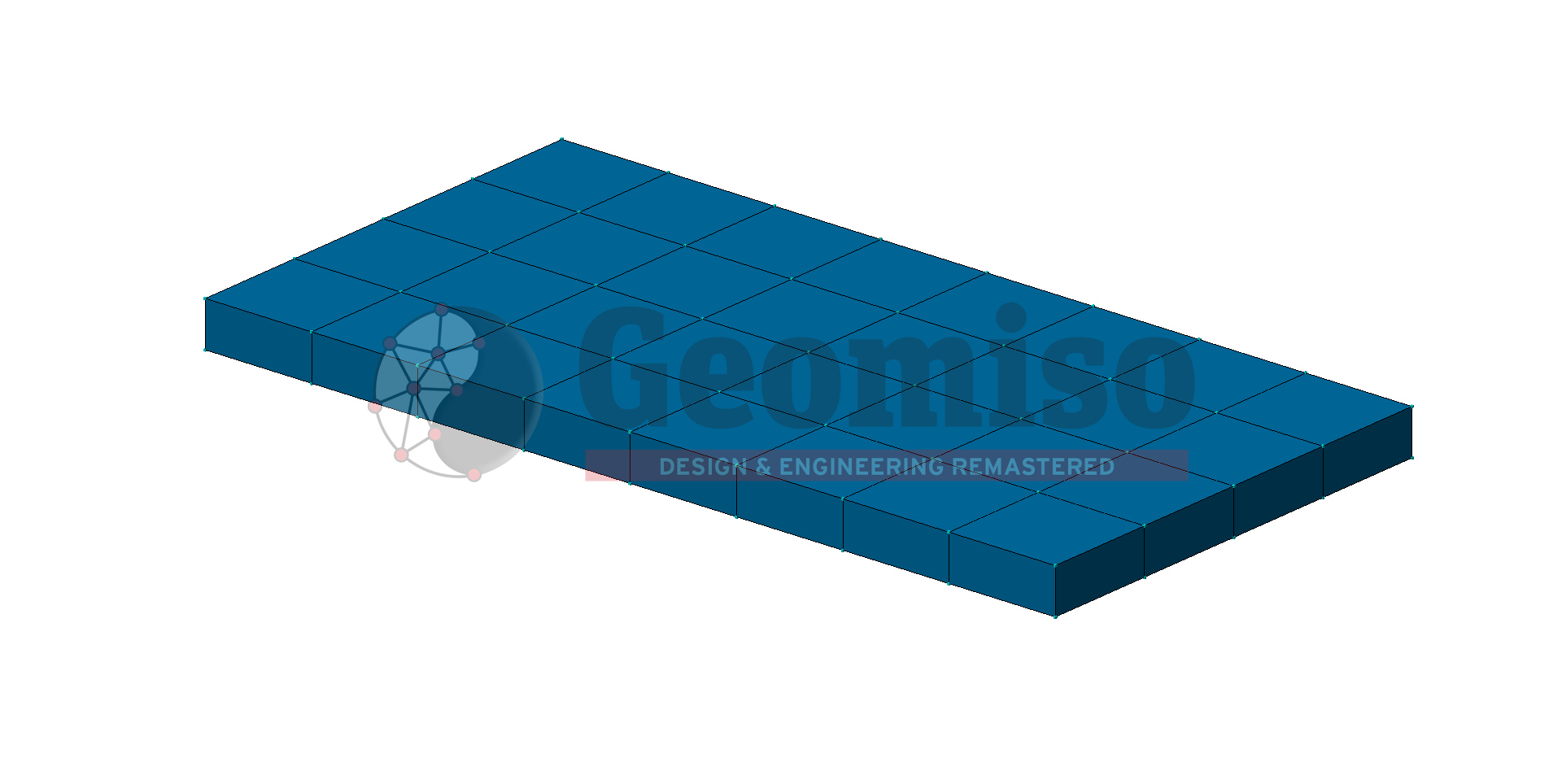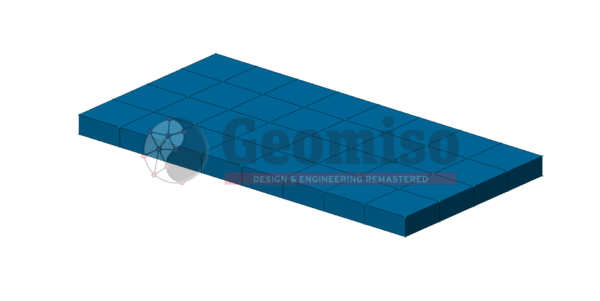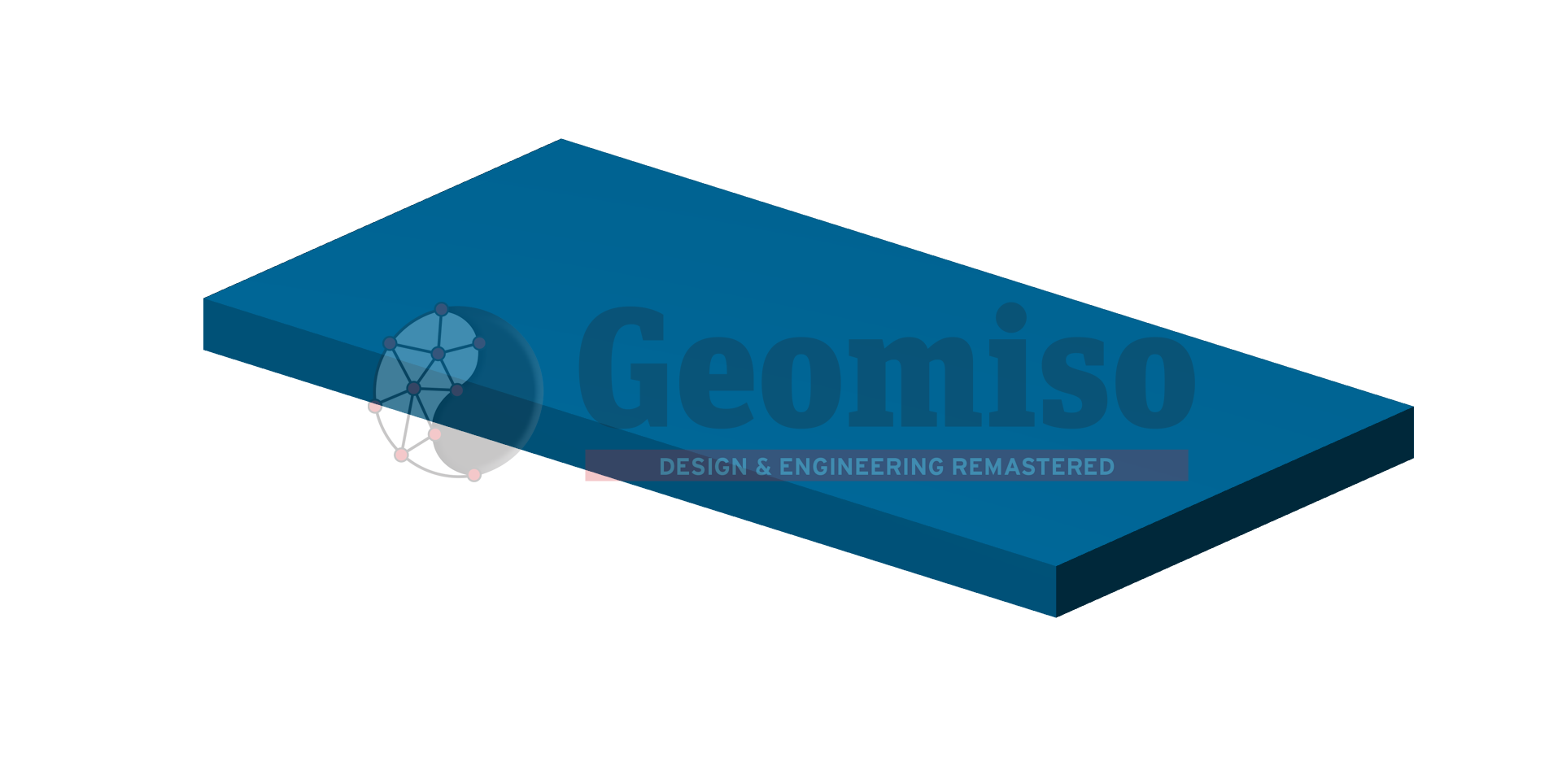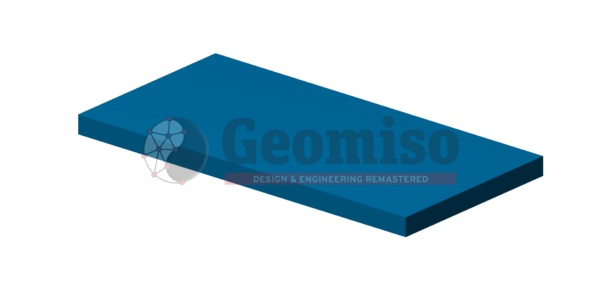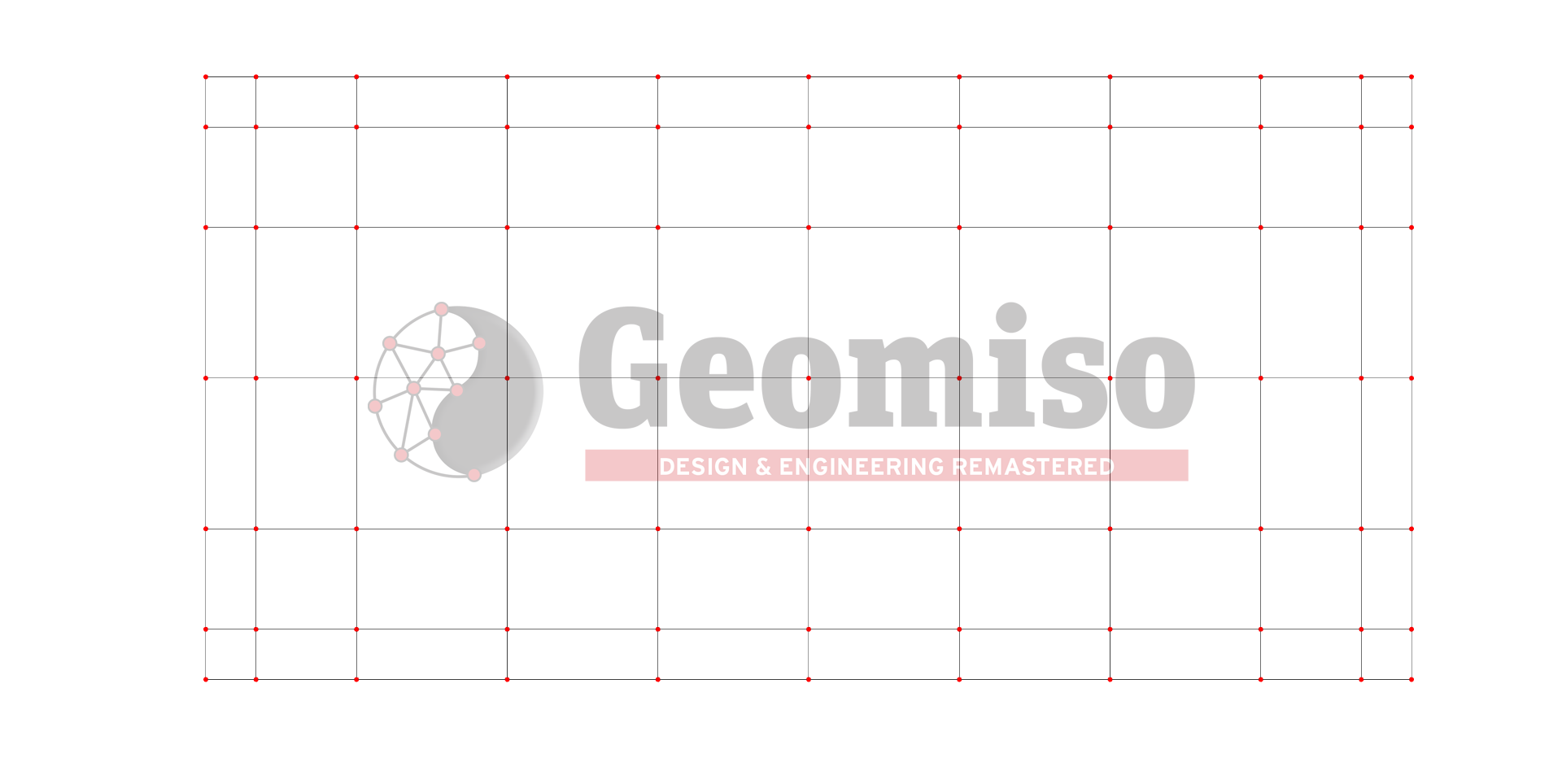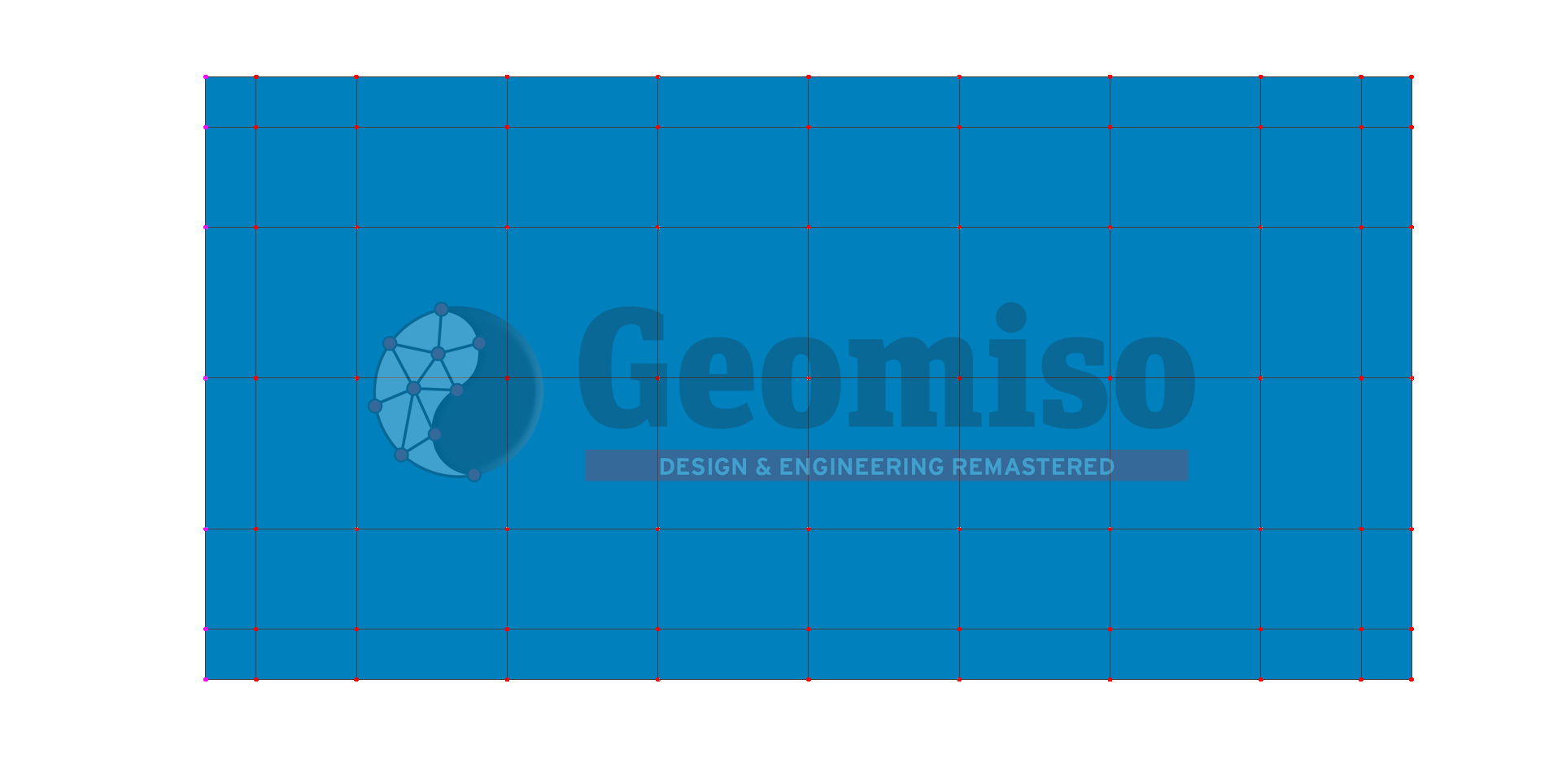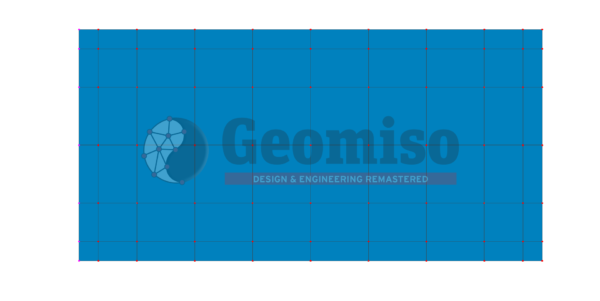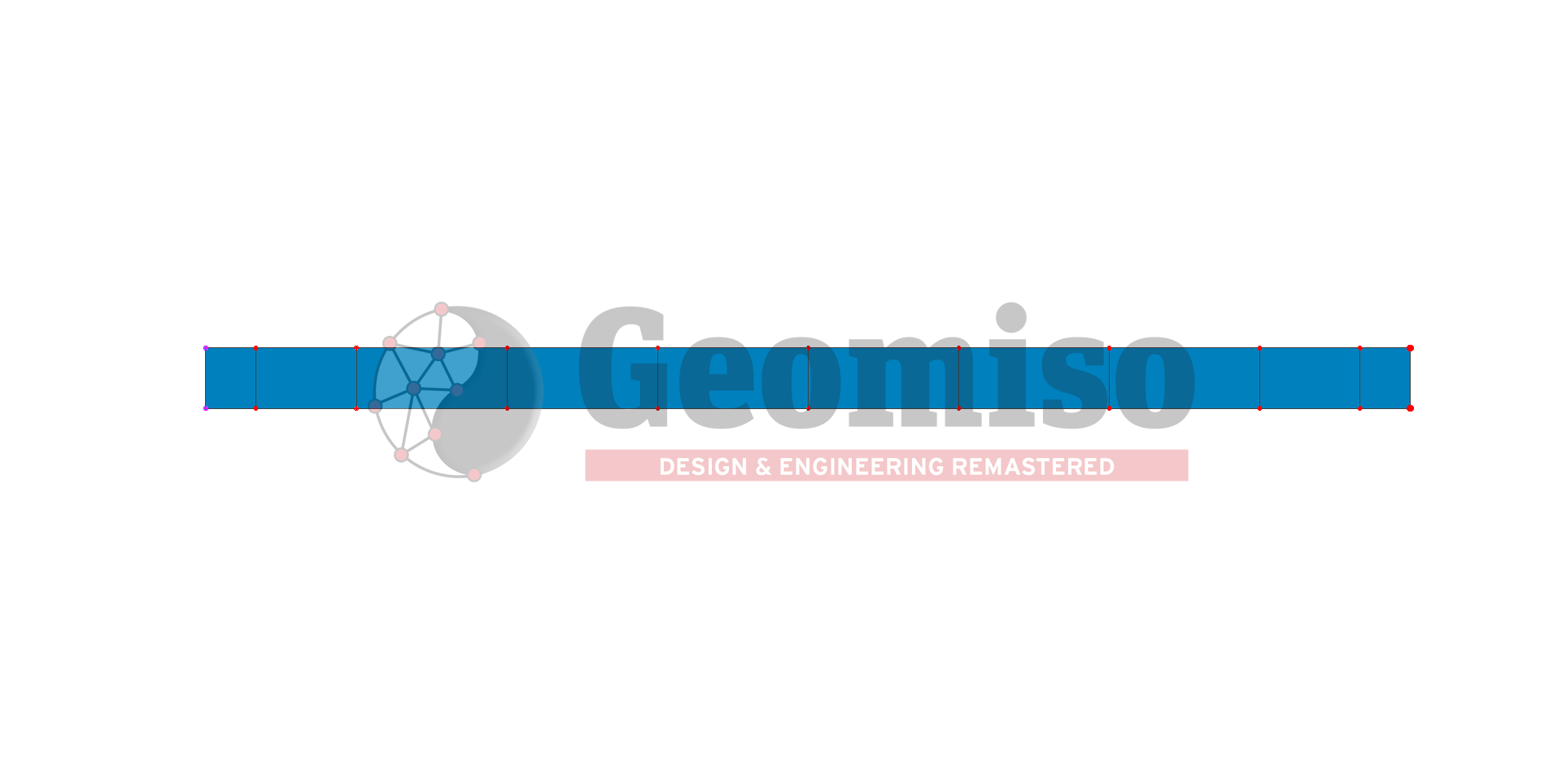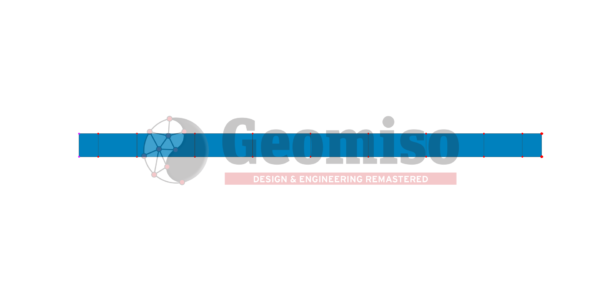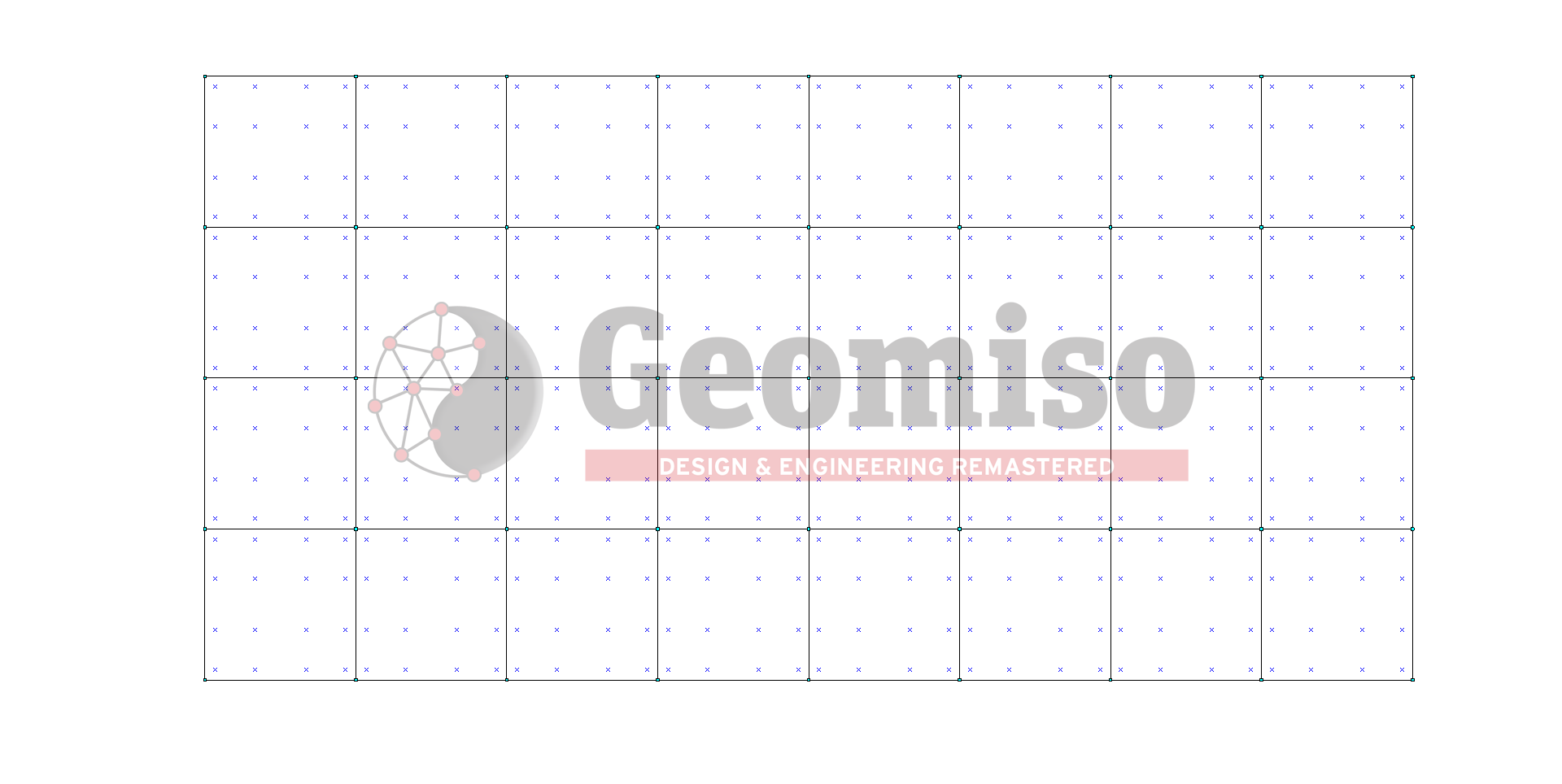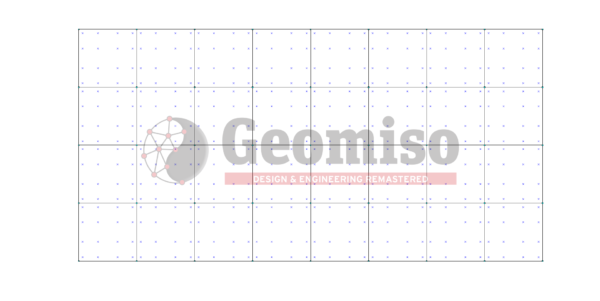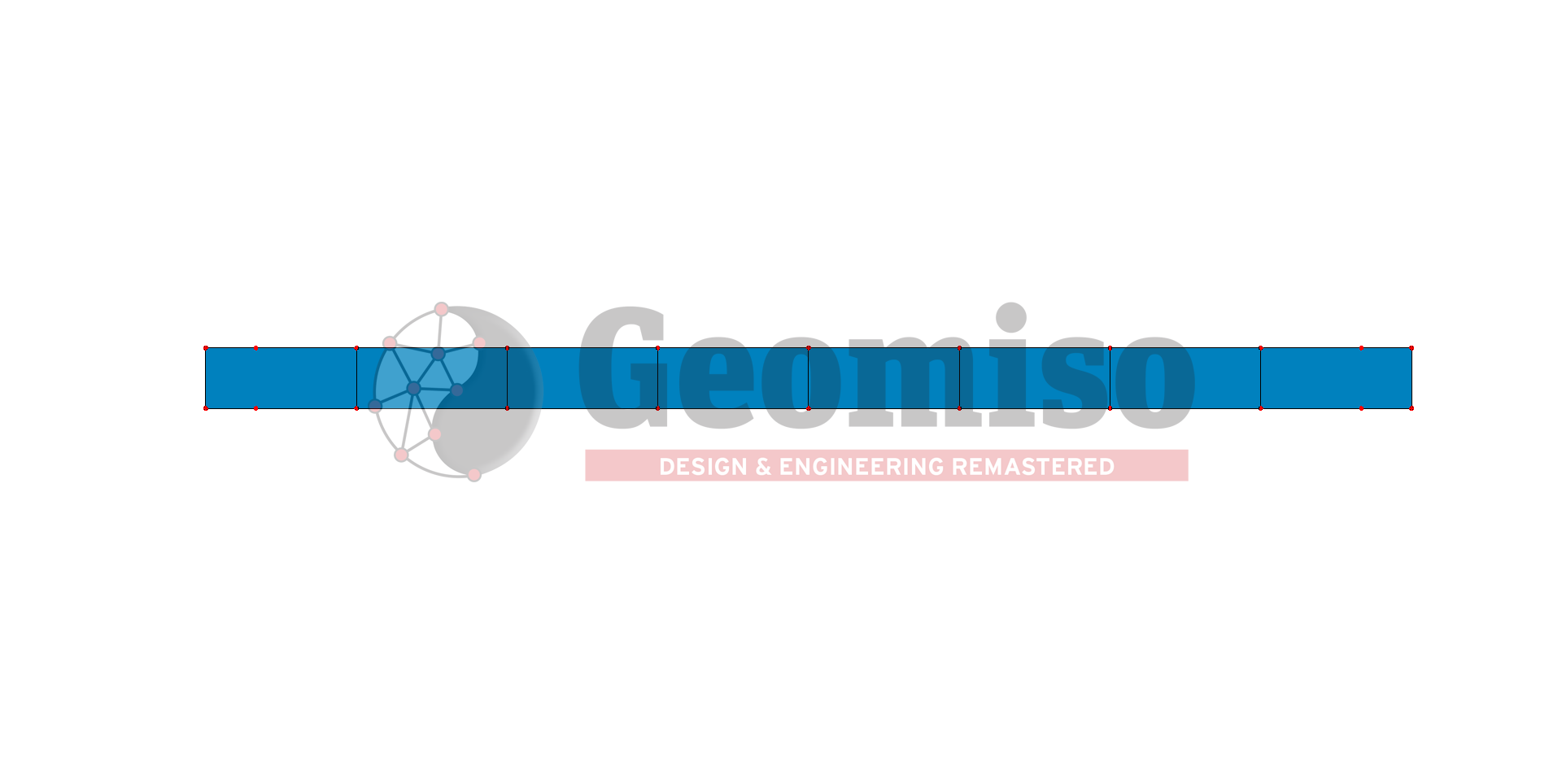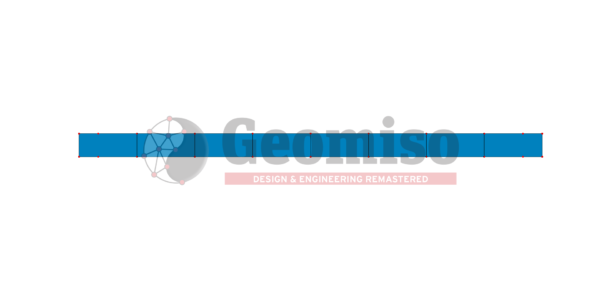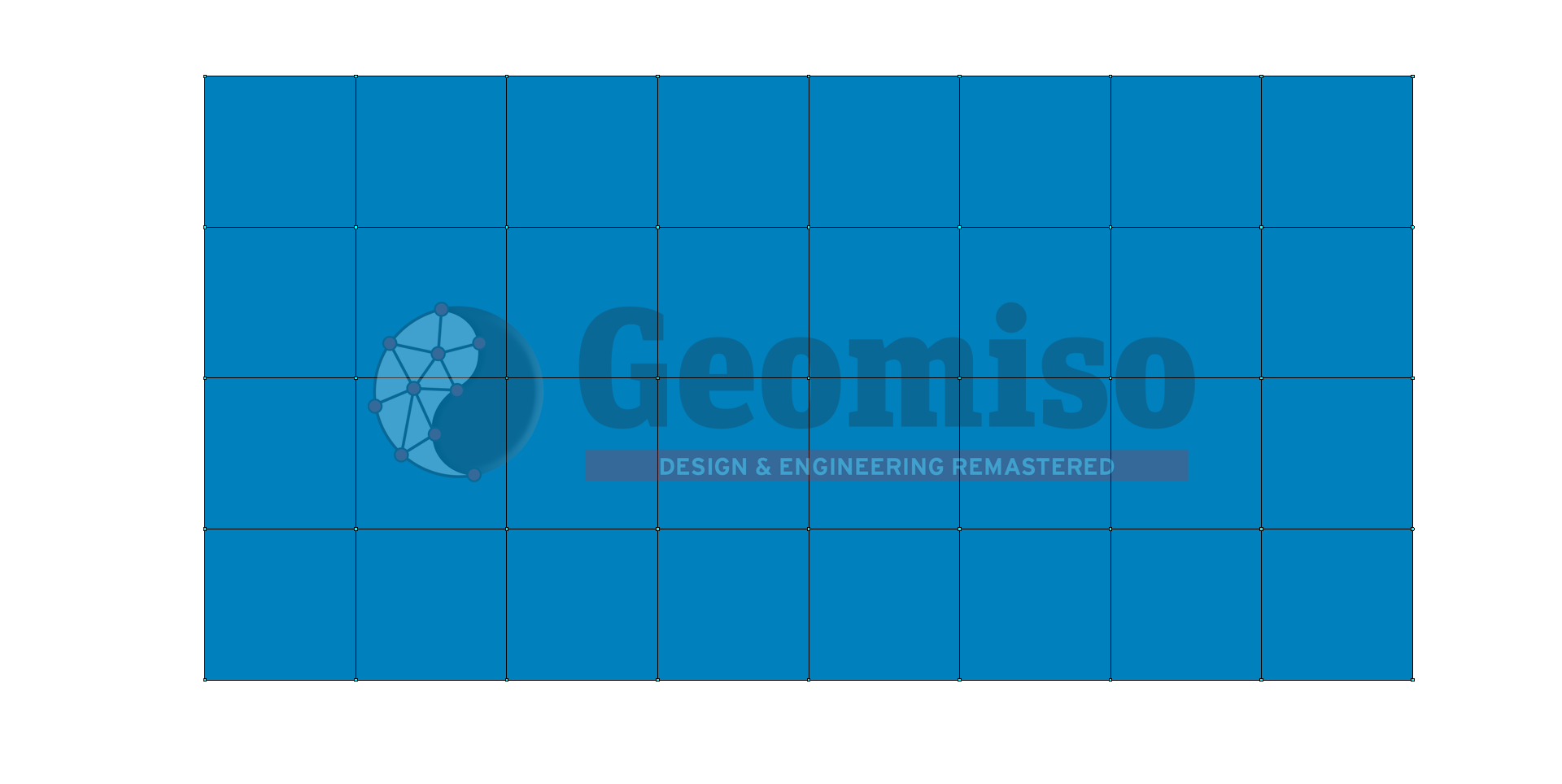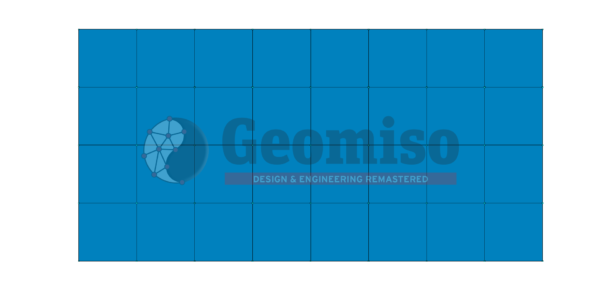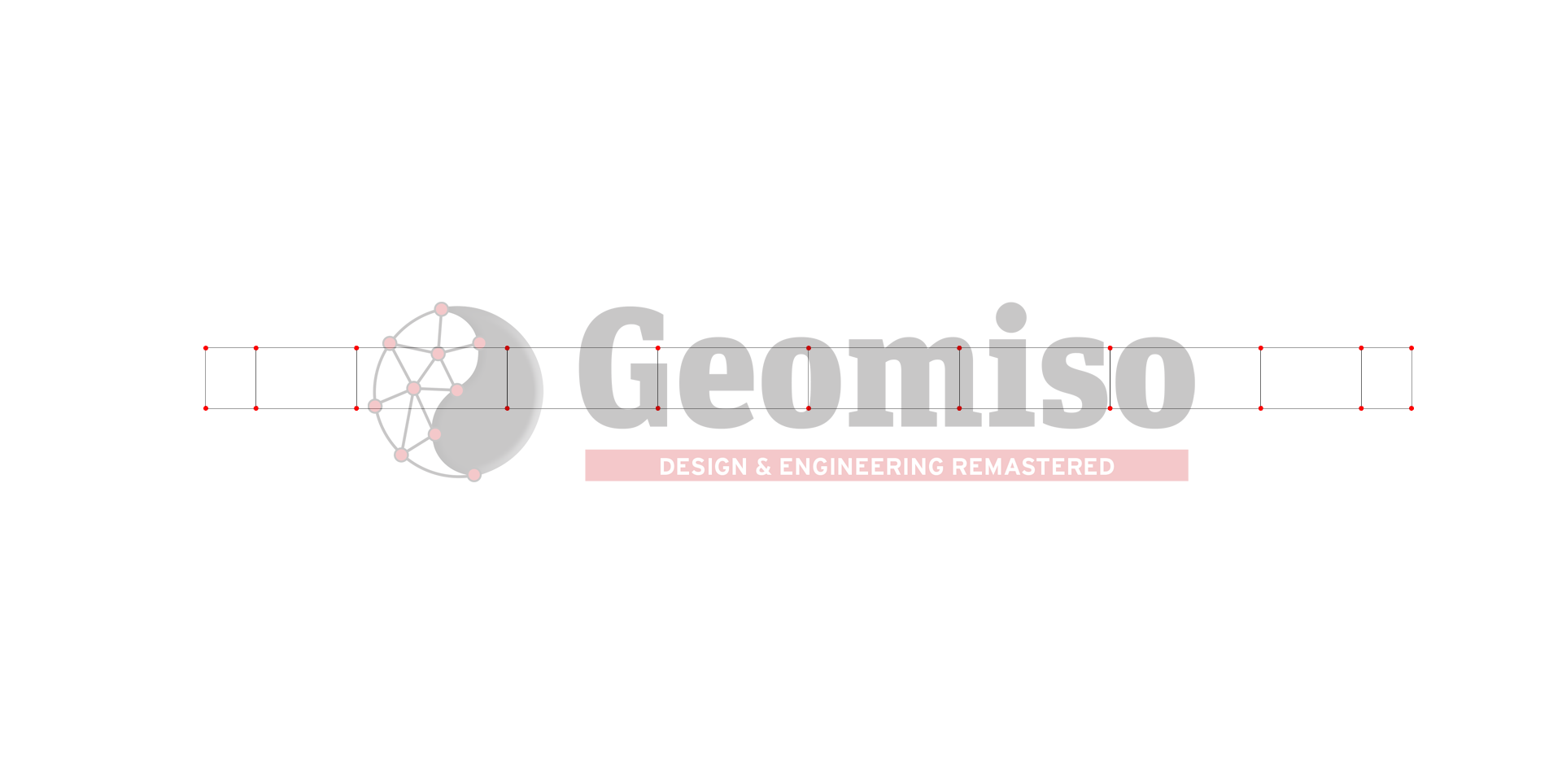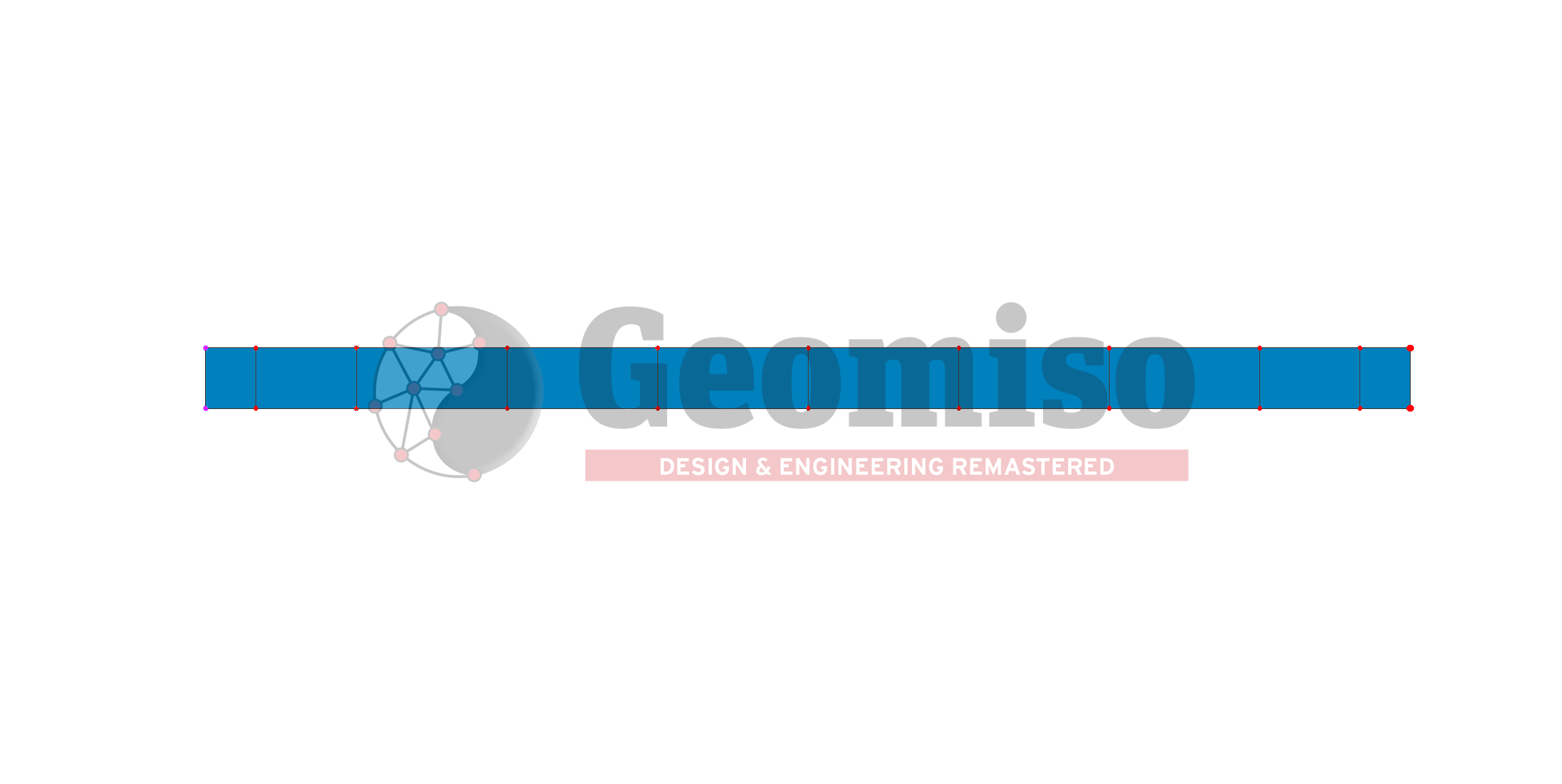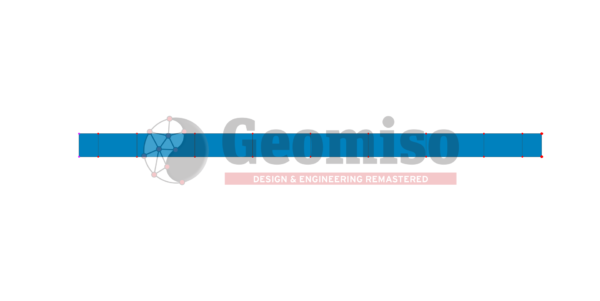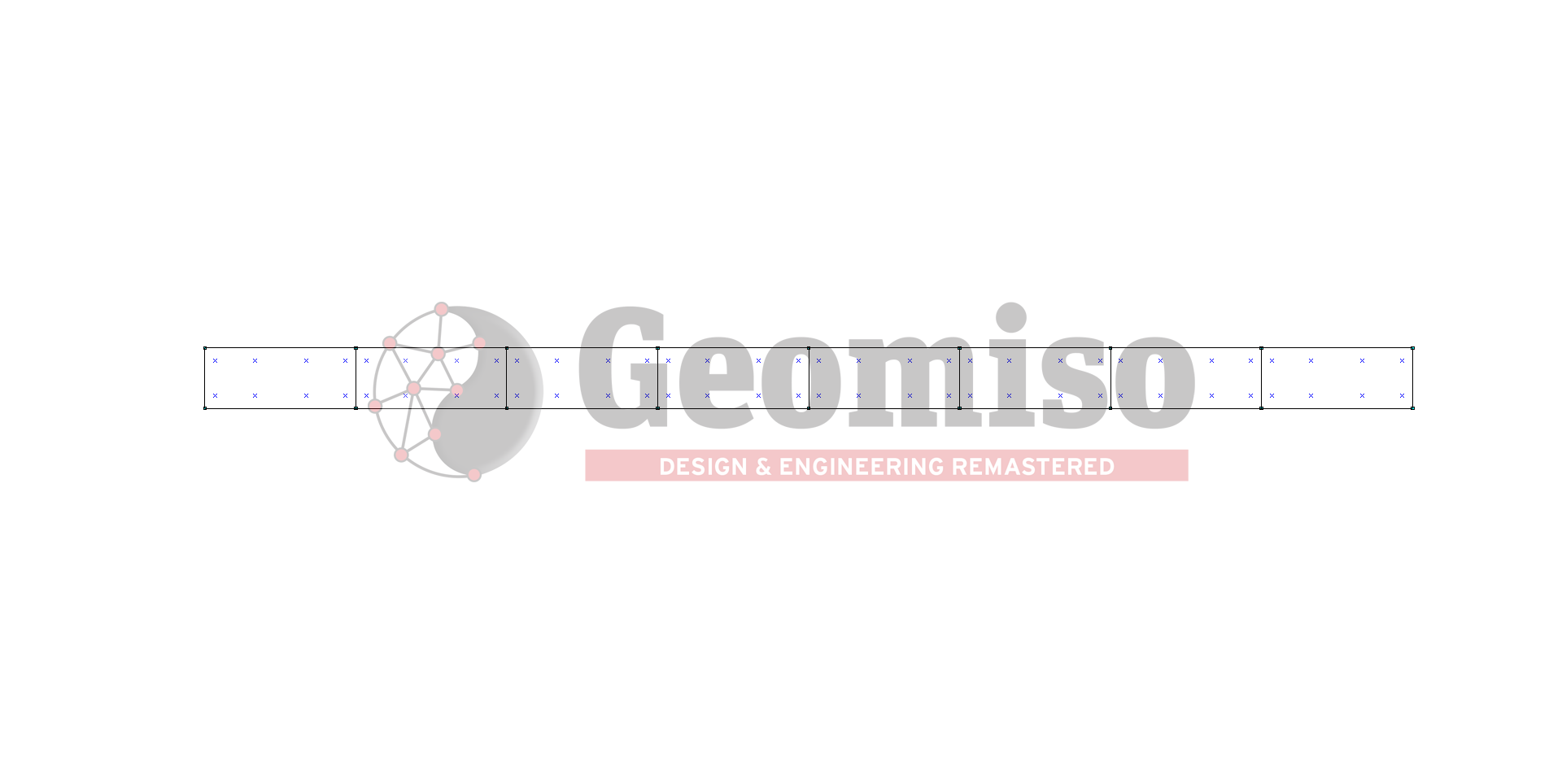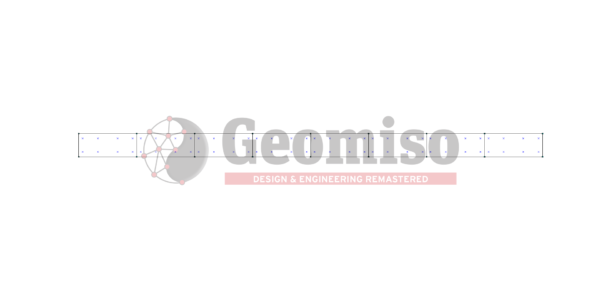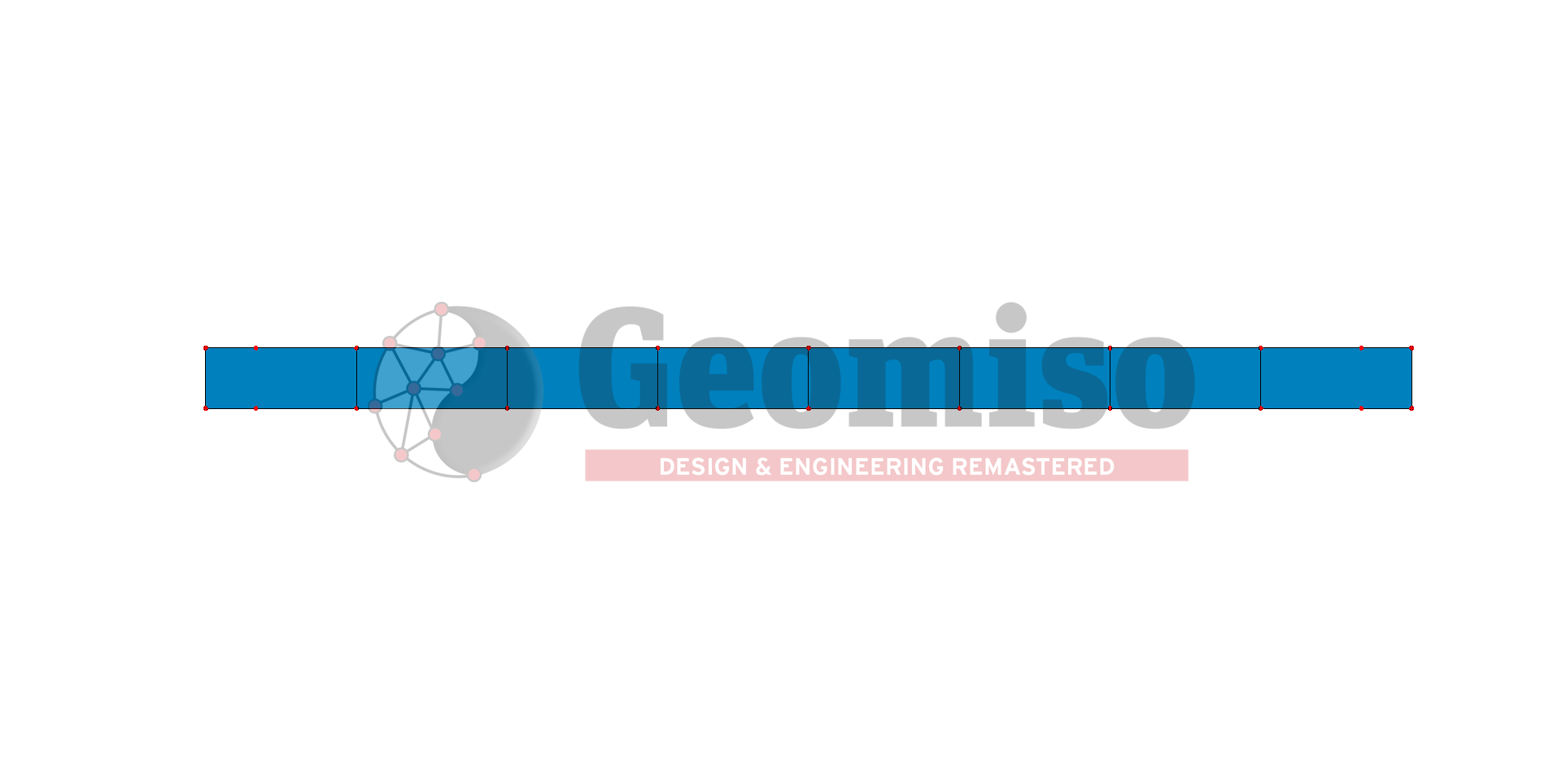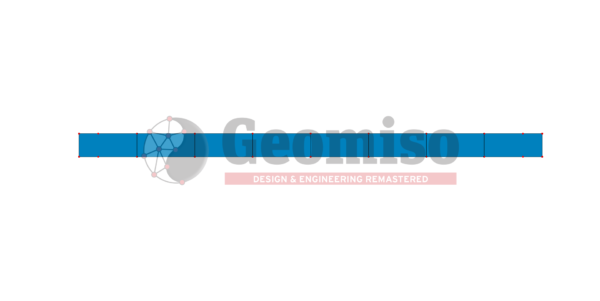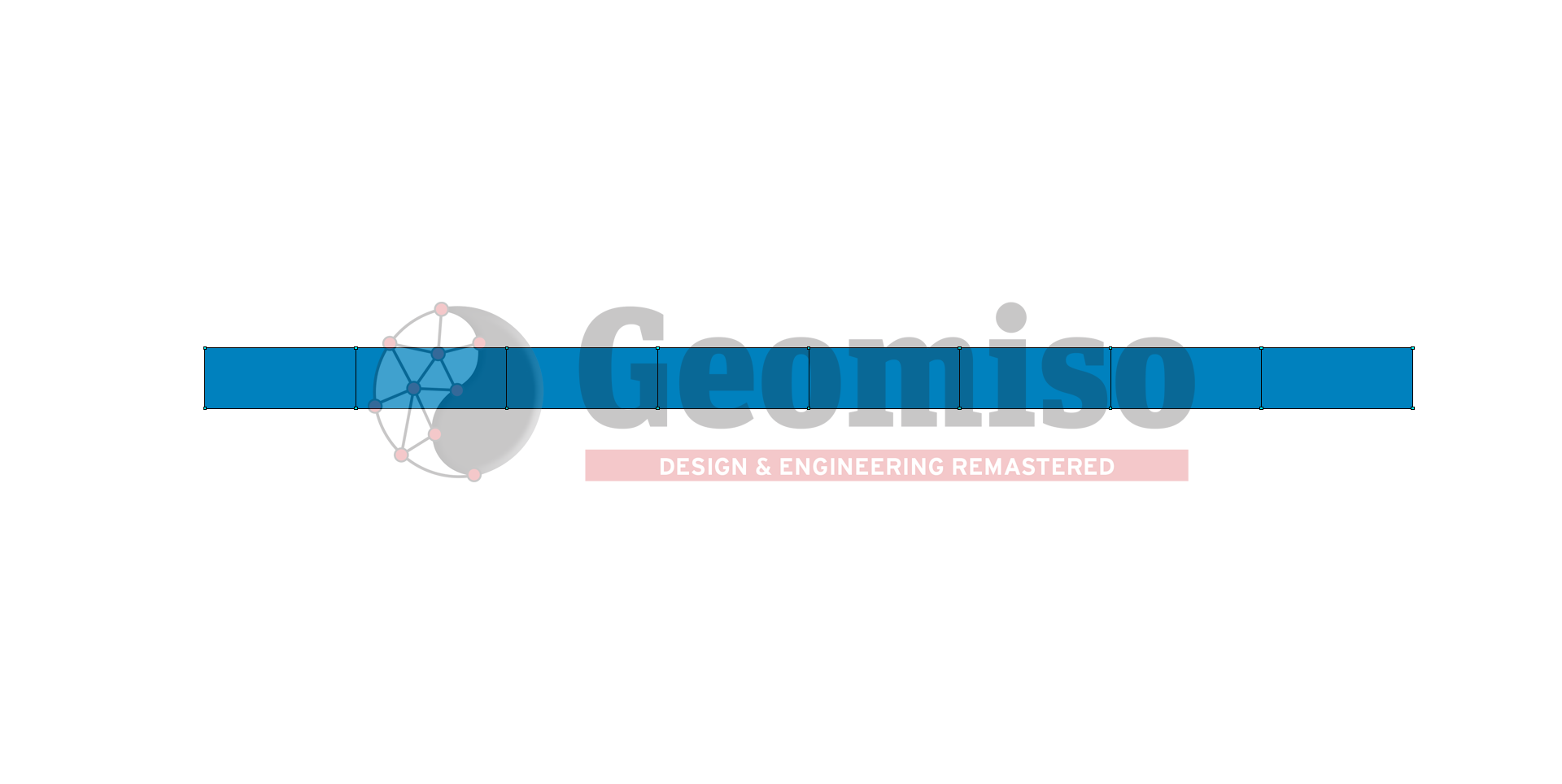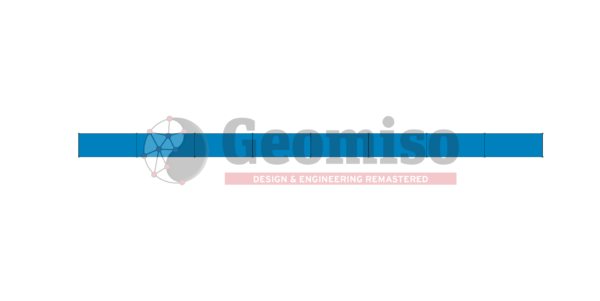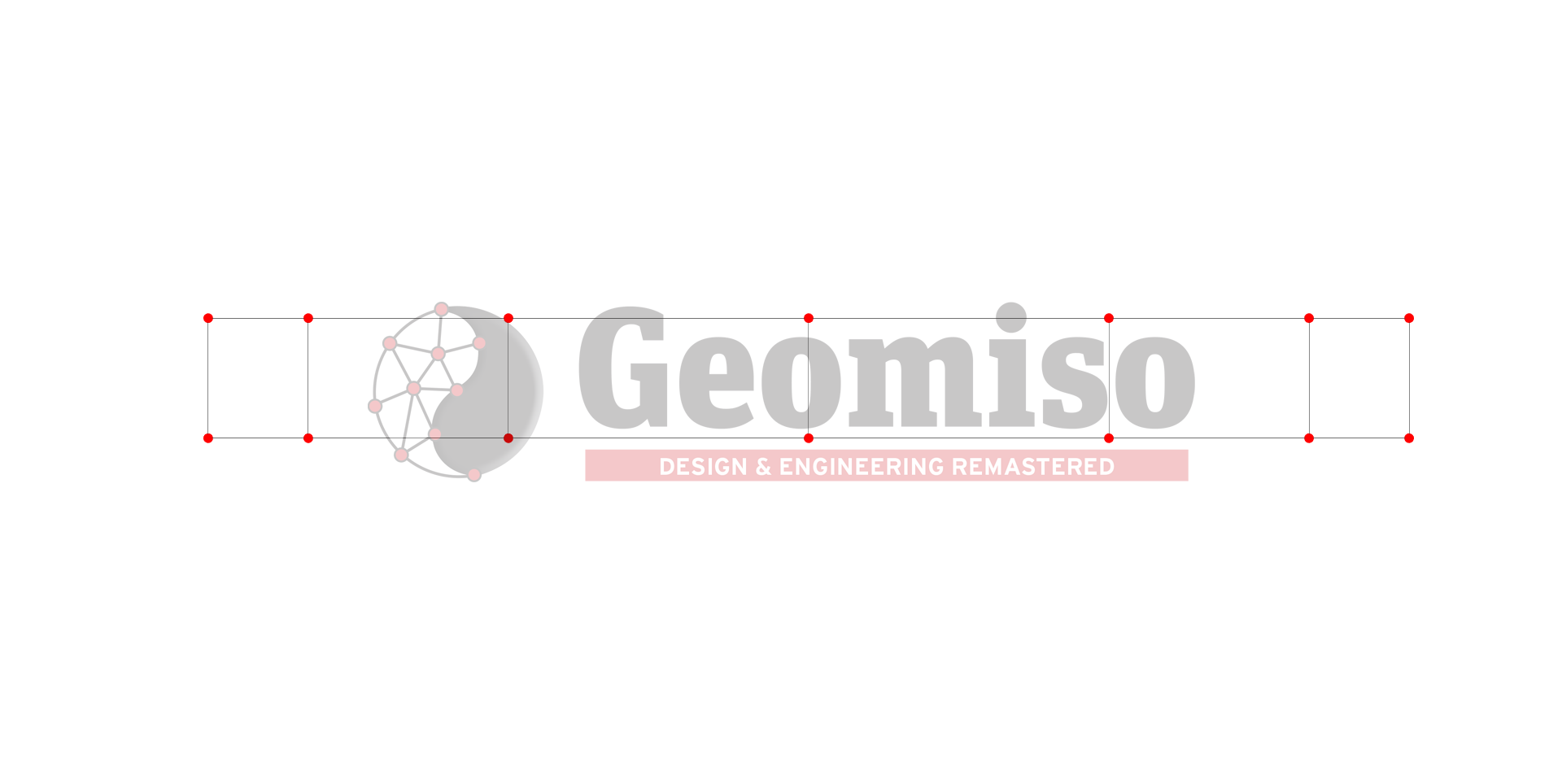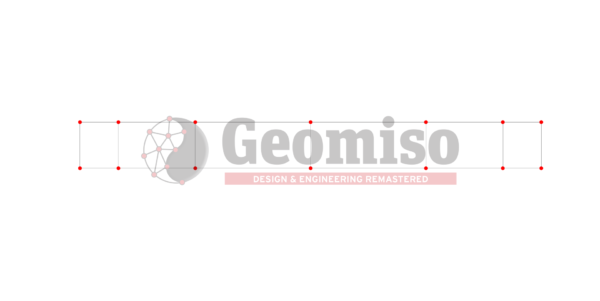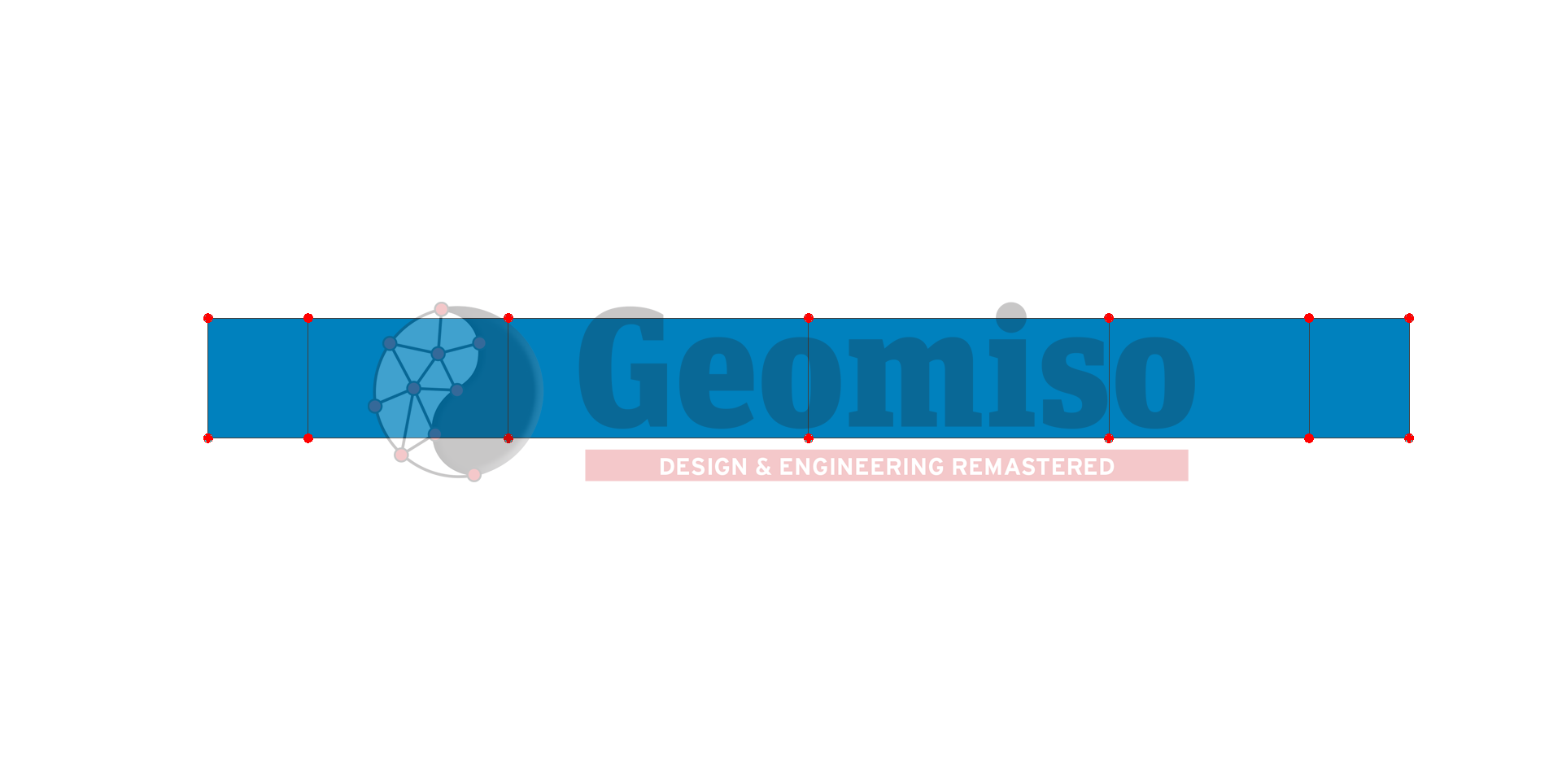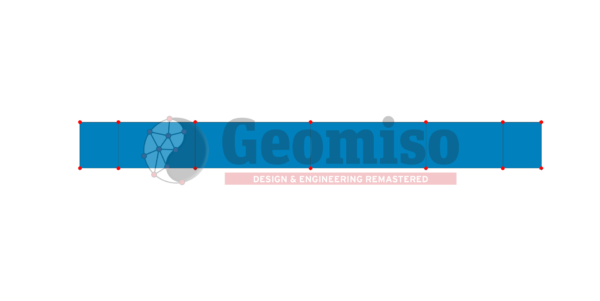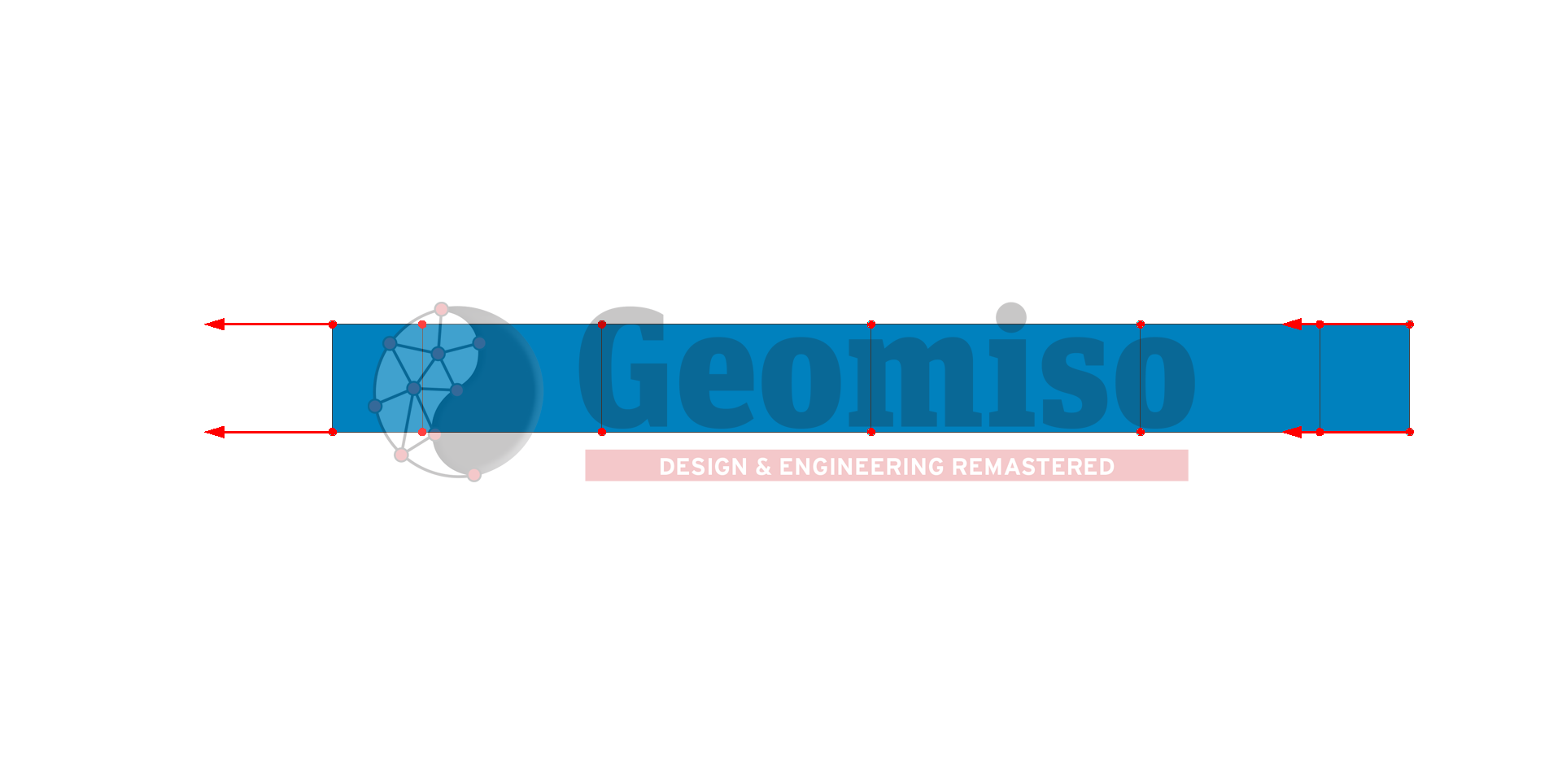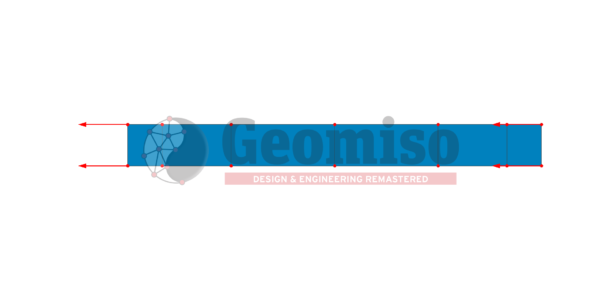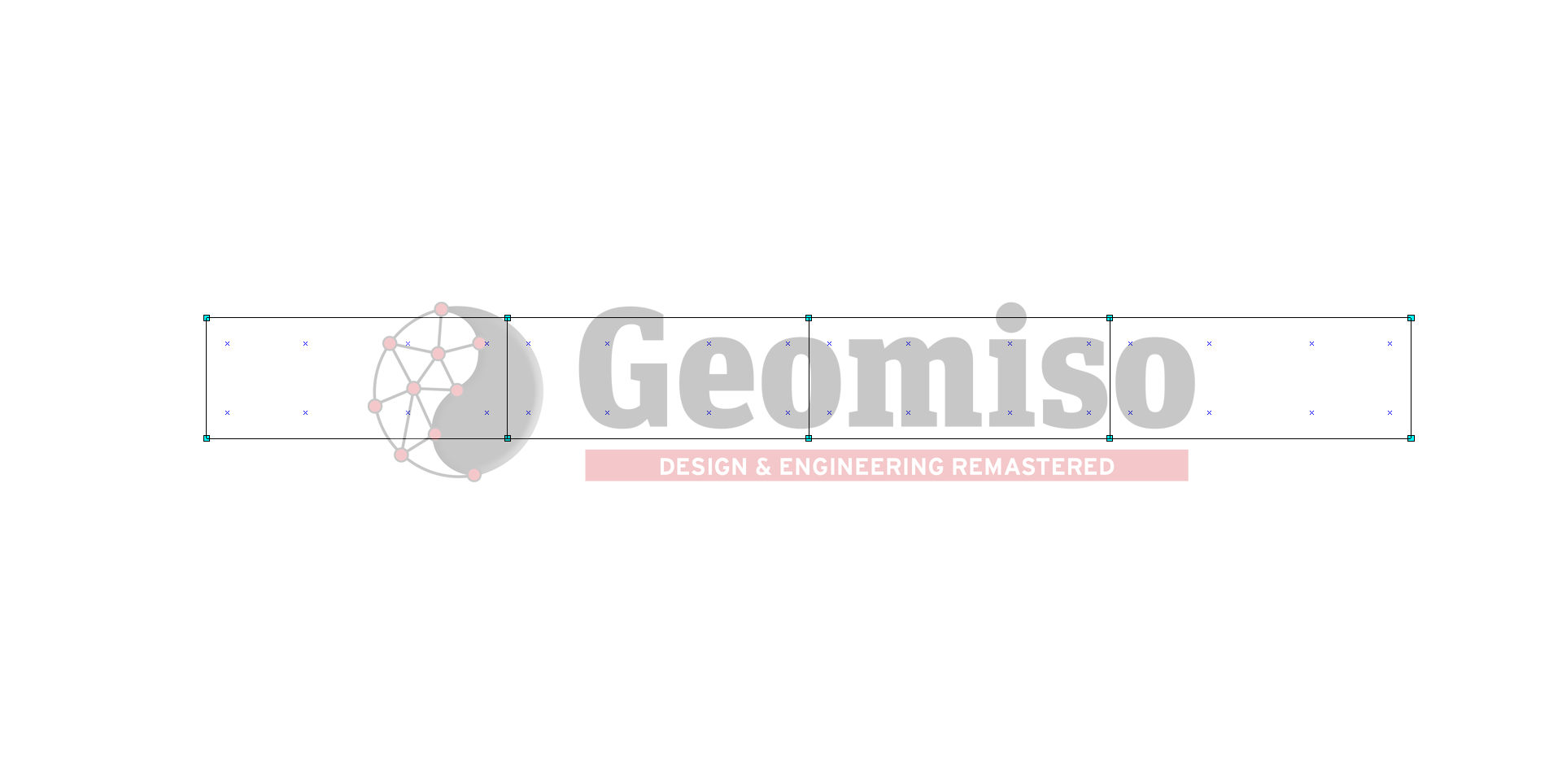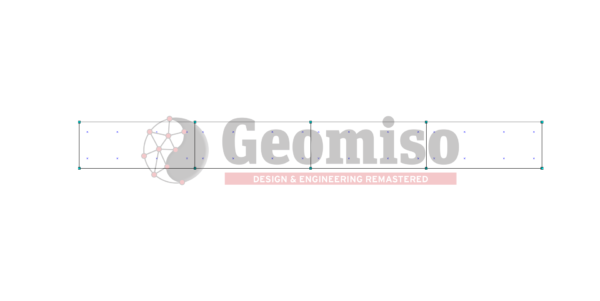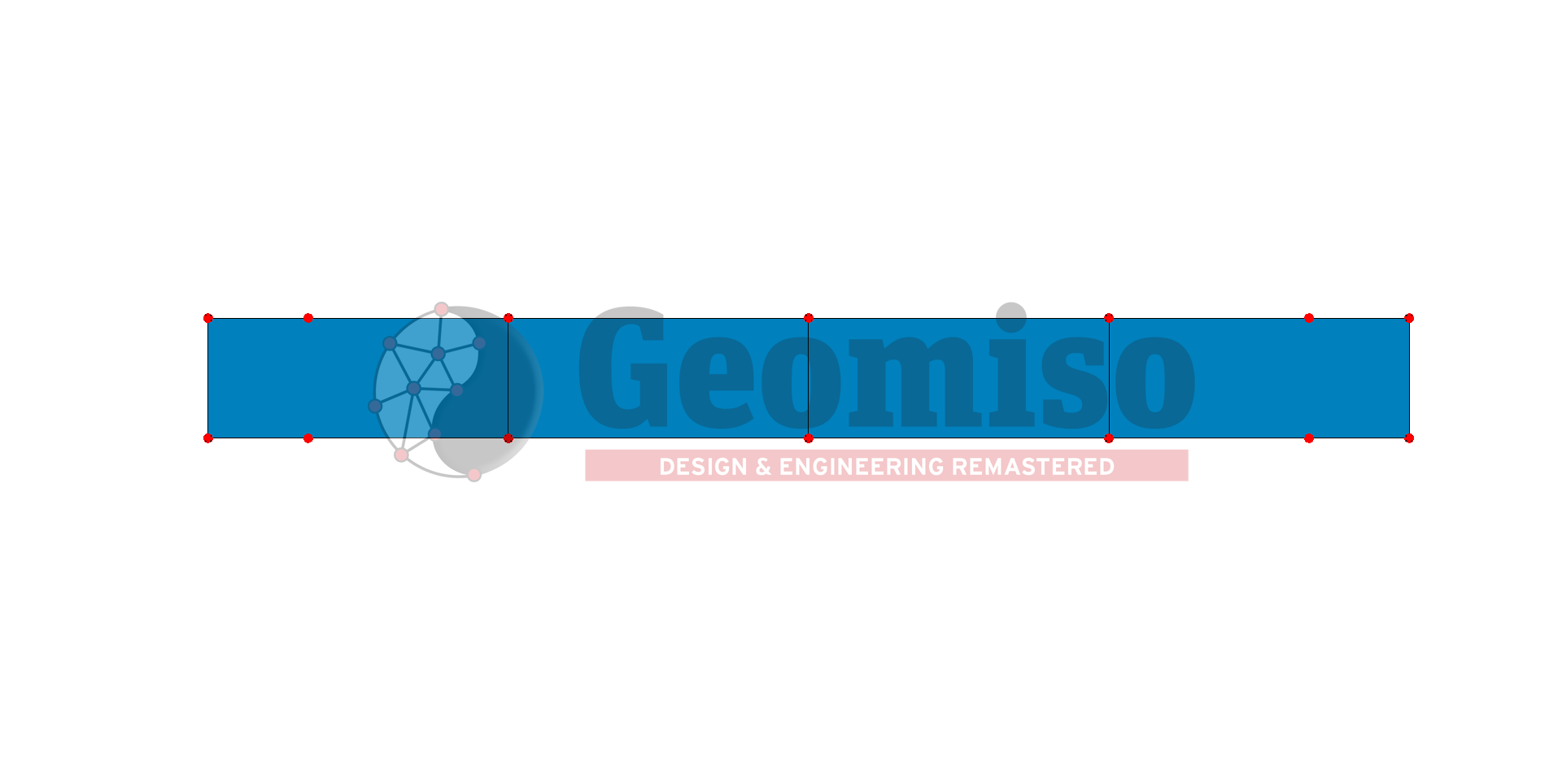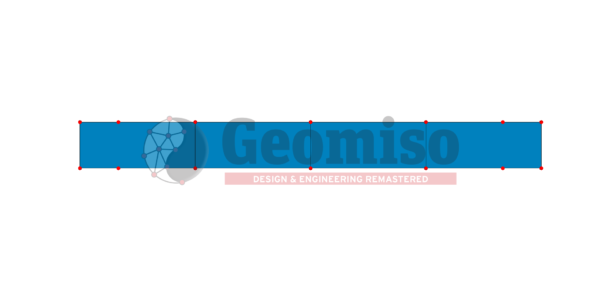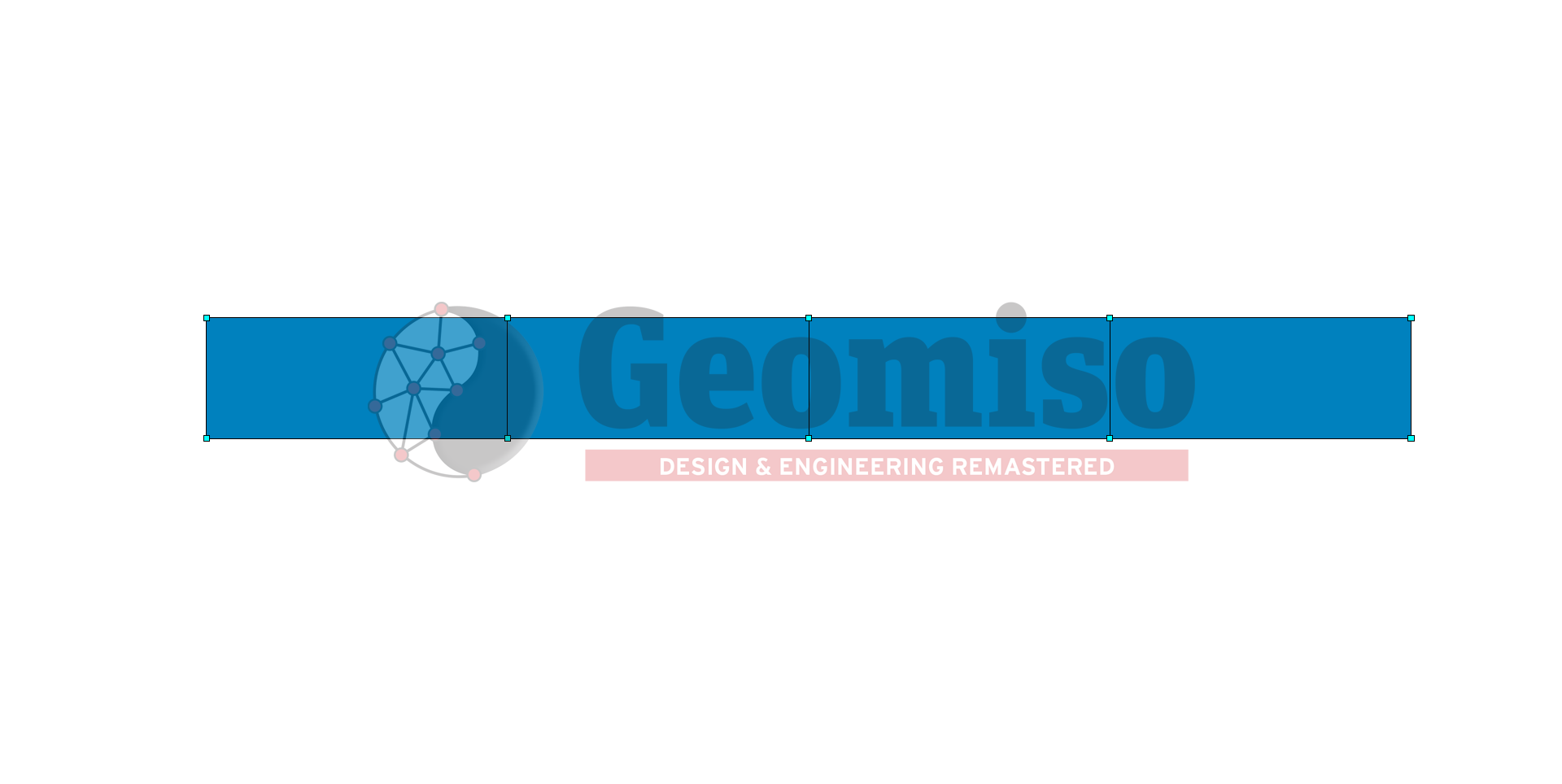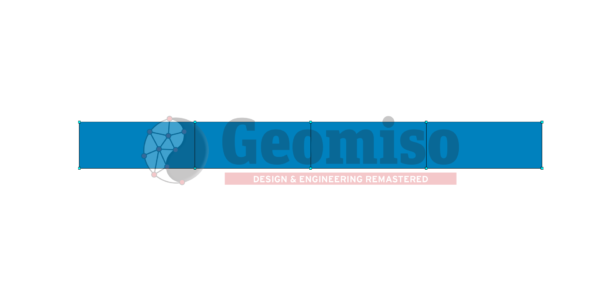# 140001. Metal plate (Contact us)

This project presents the effect of both knot span number and basis polynomial order on the numerical solution of a cantilever metal plate with homogeneous Dirichlet boundary conditions, described with NURBS and subjected to linear loading.

Categories: ,

## Description

This project presents the effect of both knot span number and basis polynomial order on the numerical solution of a cantilever metal plate with homogeneous Dirichlet boundary conditions, described with NURBS and subjected to linear loading. This parametric investigation includes a set of 9 comparisons with a total number of 20 meshes submitted to linear elastic static NURBS-based isogeometric analysis using Geomiso. The results contain pseudo-displacements (control points), Cartesian coordinates (points, Gauss points) and displacement/ strain/ stress vectors (points, Gauss points). Error estimation is based on the finest mesh which is different for each comparison, while a comparison with the respective 2D problem (130001) is conducted to estimate the errors introduced by the plane stress assumptions.

Specifications
Product ID 140001
Published Coming soon
Size MB
Product Type Project
Mesh Number 20
Geometry Rectangular parallelepiped
Material Steel
Constraint Dirichlet (homogeneous)
Load Linear
Analysis Type Isogeometric (NURBS) – Static – 3D
Material/ Geometry linearity Linear/ Linear
Quadrature Gauss-Legendre

This product includes the following files:

•  A five-page report (1 .pdf file,  MB)
•  A Microsoft Excel file with 31 worksheets (1 .xlsx file, 2.52 MB)
•  MATLAB workspaces (20 .mat files, 0.52 MB)
•  Images ( png files,  MB)

## Reviews

There are no reviews yet.